# JP4882956B2 - Image processing apparatus and image processing method - Google Patents

## Info

Publication number
JP4882956B2
JP4882956B2 JP2007273606A JP2007273606A JP4882956B2 JP 4882956 B2 JP4882956 B2 JP 4882956B2 JP 2007273606 A JP2007273606 A JP 2007273606A JP 2007273606 A JP2007273606 A JP 2007273606A JP 4882956 B2 JP4882956 B2 JP 4882956B2
Authority
JP
Japan
Prior art keywords
motion vector
plane
reduced
unit
block
Prior art date
Legal status (The legal status is an assumption and is not a legal conclusion. Google has not performed a legal analysis and makes no representation as to the accuracy of the status listed.)
Expired - Fee Related
Application number
JP2007273606A
Other languages
Japanese (ja)
Other versions
JP2009104284A (en
Inventor

Original Assignee
ソニー株式会社
Priority date (The priority date is an assumption and is not a legal conclusion. Google has not performed a legal analysis and makes no representation as to the accuracy of the date listed.)
Filing date
Publication date
Application filed by ソニー株式会社 filed Critical ソニー株式会社
Priority to JP2007273606A priority Critical patent/JP4882956B2/en
Publication of JP2009104284A publication Critical patent/JP2009104284A/en
Application granted granted Critical
Publication of JP4882956B2 publication Critical patent/JP4882956B2/en
Application status is Expired - Fee Related legal-status Critical
Anticipated expiration legal-status Critical

## Images

•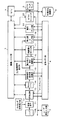•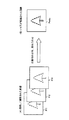•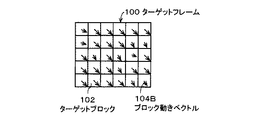•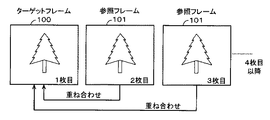•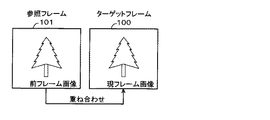•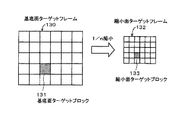••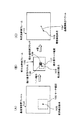•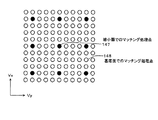•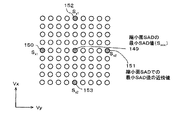•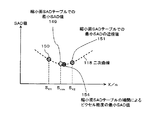•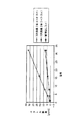•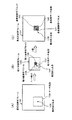•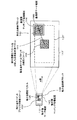•••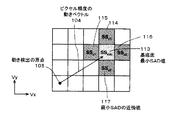••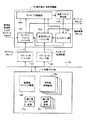•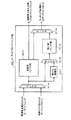•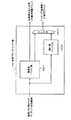••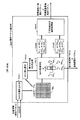•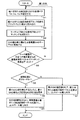•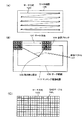•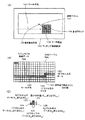•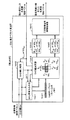•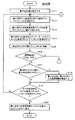•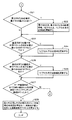••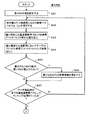•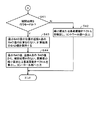••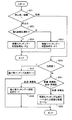•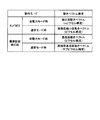•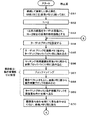•••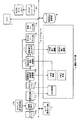•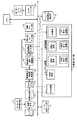•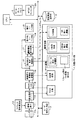••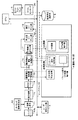••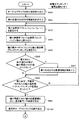•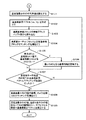•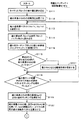•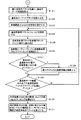•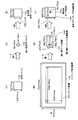•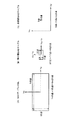•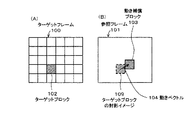••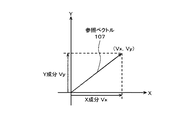•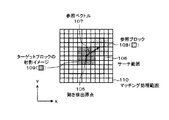•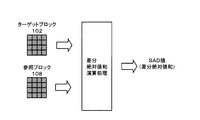•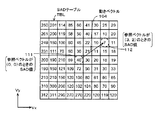## Classifications

• HELECTRICITY
• H04ELECTRIC COMMUNICATION TECHNIQUE
• H04NPICTORIAL COMMUNICATION, e.g. TELEVISION
• H04N5/00Details of television systems
• H04N5/14Picture signal circuitry for video frequency region
• H04N5/144Movement detection
• H04N5/145Movement estimation
• GPHYSICS
• G06COMPUTING; CALCULATING; COUNTING
• G06TIMAGE DATA PROCESSING OR GENERATION, IN GENERAL
• G06T7/00Image analysis
• G06T7/20Analysis of motion
• G06T7/223Analysis of motion using block-matching
• HELECTRICITY
• H04ELECTRIC COMMUNICATION TECHNIQUE
• H04NPICTORIAL COMMUNICATION, e.g. TELEVISION
• H04N19/00Methods or arrangements for coding, decoding, compressing or decompressing digital video signals
• H04N19/10Methods or arrangements for coding, decoding, compressing or decompressing digital video signals using adaptive coding
• H04N19/102Methods or arrangements for coding, decoding, compressing or decompressing digital video signals using adaptive coding characterised by the element, parameter or selection affected or controlled by the adaptive coding
• HELECTRICITY
• H04ELECTRIC COMMUNICATION TECHNIQUE
• H04NPICTORIAL COMMUNICATION, e.g. TELEVISION
• H04N19/00Methods or arrangements for coding, decoding, compressing or decompressing digital video signals
• H04N19/10Methods or arrangements for coding, decoding, compressing or decompressing digital video signals using adaptive coding
• H04N19/134Methods or arrangements for coding, decoding, compressing or decompressing digital video signals using adaptive coding characterised by the element, parameter or criterion affecting or controlling the adaptive coding
• H04N19/156Availability of hardware or computational resources, e.g. encoding based on power-saving criteria
• HELECTRICITY
• H04ELECTRIC COMMUNICATION TECHNIQUE
• H04NPICTORIAL COMMUNICATION, e.g. TELEVISION
• H04N19/00Methods or arrangements for coding, decoding, compressing or decompressing digital video signals
• H04N19/10Methods or arrangements for coding, decoding, compressing or decompressing digital video signals using adaptive coding
• H04N19/169Methods or arrangements for coding, decoding, compressing or decompressing digital video signals using adaptive coding characterised by the coding unit, i.e. the structural portion or semantic portion of the video signal being the object or the subject of the adaptive coding
• H04N19/17Methods or arrangements for coding, decoding, compressing or decompressing digital video signals using adaptive coding characterised by the coding unit, i.e. the structural portion or semantic portion of the video signal being the object or the subject of the adaptive coding the unit being an image region, e.g. an object
• H04N19/172Methods or arrangements for coding, decoding, compressing or decompressing digital video signals using adaptive coding characterised by the coding unit, i.e. the structural portion or semantic portion of the video signal being the object or the subject of the adaptive coding the unit being an image region, e.g. an object the region being a picture, frame or field
• HELECTRICITY
• H04ELECTRIC COMMUNICATION TECHNIQUE
• H04NPICTORIAL COMMUNICATION, e.g. TELEVISION
• H04N19/00Methods or arrangements for coding, decoding, compressing or decompressing digital video signals
• H04N19/50Methods or arrangements for coding, decoding, compressing or decompressing digital video signals using predictive coding
• H04N19/503Methods or arrangements for coding, decoding, compressing or decompressing digital video signals using predictive coding involving temporal prediction
• H04N19/51Motion estimation or motion compensation
• H04N19/513Processing of motion vectors
• H04N19/521Processing of motion vectors for estimating the reliability of the determined motion vectors or motion vector field, e.g. for smoothing the motion vector field or for correcting motion vectors
• HELECTRICITY
• H04ELECTRIC COMMUNICATION TECHNIQUE
• H04NPICTORIAL COMMUNICATION, e.g. TELEVISION
• H04N19/00Methods or arrangements for coding, decoding, compressing or decompressing digital video signals
• H04N19/50Methods or arrangements for coding, decoding, compressing or decompressing digital video signals using predictive coding
• H04N19/503Methods or arrangements for coding, decoding, compressing or decompressing digital video signals using predictive coding involving temporal prediction
• H04N19/51Motion estimation or motion compensation
• H04N19/523Motion estimation or motion compensation with sub-pixel accuracy
• HELECTRICITY
• H04ELECTRIC COMMUNICATION TECHNIQUE
• H04NPICTORIAL COMMUNICATION, e.g. TELEVISION
• H04N19/00Methods or arrangements for coding, decoding, compressing or decompressing digital video signals
• H04N19/50Methods or arrangements for coding, decoding, compressing or decompressing digital video signals using predictive coding
• H04N19/503Methods or arrangements for coding, decoding, compressing or decompressing digital video signals using predictive coding involving temporal prediction
• H04N19/51Motion estimation or motion compensation
• H04N19/53Multi-resolution motion estimation; Hierarchical motion estimation
• HELECTRICITY
• H04ELECTRIC COMMUNICATION TECHNIQUE
• H04NPICTORIAL COMMUNICATION, e.g. TELEVISION
• H04N19/00Methods or arrangements for coding, decoding, compressing or decompressing digital video signals
• H04N19/50Methods or arrangements for coding, decoding, compressing or decompressing digital video signals using predictive coding
• H04N19/59Methods or arrangements for coding, decoding, compressing or decompressing digital video signals using predictive coding involving spatial sub-sampling or interpolation, e.g. alteration of picture size or resolution
• HELECTRICITY
• H04ELECTRIC COMMUNICATION TECHNIQUE
• H04NPICTORIAL COMMUNICATION, e.g. TELEVISION
• H04N19/00Methods or arrangements for coding, decoding, compressing or decompressing digital video signals
• H04N19/60Methods or arrangements for coding, decoding, compressing or decompressing digital video signals using transform coding
• H04N19/61Methods or arrangements for coding, decoding, compressing or decompressing digital video signals using transform coding in combination with predictive coding
• HELECTRICITY
• H04ELECTRIC COMMUNICATION TECHNIQUE
• H04NPICTORIAL COMMUNICATION, e.g. TELEVISION
• H04N19/00Methods or arrangements for coding, decoding, compressing or decompressing digital video signals
• H04N19/80Details of filtering operations specially adapted for video compression, e.g. for pixel interpolation
• H04N19/82Details of filtering operations specially adapted for video compression, e.g. for pixel interpolation involving filtering within a prediction loop
• HELECTRICITY
• H04ELECTRIC COMMUNICATION TECHNIQUE
• H04NPICTORIAL COMMUNICATION, e.g. TELEVISION
• H04N19/00Methods or arrangements for coding, decoding, compressing or decompressing digital video signals
• H04N19/85Methods or arrangements for coding, decoding, compressing or decompressing digital video signals using pre-processing or post-processing specially adapted for video compression
• GPHYSICS
• G06COMPUTING; CALCULATING; COUNTING
• G06TIMAGE DATA PROCESSING OR GENERATION, IN GENERAL
• G06T2207/00Indexing scheme for image analysis or image enhancement
• G06T2207/20Special algorithmic details
• G06T2207/20212Image combination
• G06T2207/20216Image averaging

## Description

The present invention relates to an image processing apparatus and an image processing method for detecting a motion vector between two different screens. In this specification, the screen means an image that is composed of image data for one frame or one field and is displayed on the display as one image.

A block matching method for obtaining a motion vector between two screens from image information itself is an old technology. Development has been centered on pan / tilt detection of TV cameras, subject tracking, moving picture experts group (MPEG) video coding, and sensorless camera shake correction by superimposing images since the 1990s. Applications such as noise reduction (Noise Reduction: hereinafter referred to as NR) at the time of low-illuminance photography are being promoted.

In block matching, a motion vector between two screens between a reference screen that is a target screen and an original screen (referred to as a target screen) that is a source of motion of the reference screen is a block of a rectangular area having a predetermined size. Is calculated by calculating the correlation between the reference screen and the original screen. When the original screen is temporally before the reference screen (for example, in the case of motion detection in MPEG), and when the reference screen is temporally prior to the original screen (for example, described later) Both in the case of noise reduction by superimposing image frames to be performed).

As described above, in this specification, the screen means an image composed of image data of one frame or one field, but for the convenience of the following description in this specification, the screen is composed of one frame. As an example, the screen is referred to as a frame. Therefore, the reference screen is referred to as a reference frame, and the original screen is referred to as an original frame.

FIGS. 51 to 56 are diagrams for explaining the outline of conventional block matching. In the block matching method described here, for example, as shown in FIG. 51 (A), an original frame (target frame) 100 having a predetermined size composed of a plurality of pixels in the horizontal direction and a plurality of lines in the vertical direction, respectively. Divide into rectangular areas (called blocks). Each of the plurality of blocks 102 in the target frame is referred to as a target block.

In block matching, a block having a high correlation with the target block 102 is searched from the reference frame 101. As a result of this search, the block 103 (see FIG. 51B) detected in the reference frame 101 as having the highest correlation is referred to as a motion compensation block. Further, the amount of positional deviation between the target block 102 and the motion compensation block 103 is referred to as a motion vector (see reference numeral 104 in FIG. 51B).

A motion vector 104 corresponding to a position shift (including a position shift amount and a position shift direction) between the target block 102 and the motion compensation block 103 is the same as the position of each target block 102 of the target frame 100 in the reference frame 101. Assuming that the projection image block 109 of the target block 102 is assumed as the position, a positional shift between the position of the projection image block 109 of the target block (for example, the center position) and the position of the motion compensation block 103 (for example, the center position). It also has a positional deviation amount and a positional deviation direction component.

An outline of the block matching process will be described. As shown by a dotted line in FIG. 52, in the reference frame 101, a projected image block 109 of the target block is assumed at the same position as the position of each target block 102 of the target frame 100, and the center of the projected image block 109 of this target block is assumed. The coordinates are set as a motion detection origin 105. Then, assuming that the motion vector 104 exists within a certain range from the motion detection origin 105, a predetermined range centered on the motion detection origin 105 is set as the search range 106 (see the one-dot chain line in FIG. 52). .

Next, a block (referred to as a reference block) 108 having the same size as the target block 102 is set on the reference screen. The position of the reference block 108 is moved within the search range 106 in units of one pixel or a plurality of pixels, for example, in the horizontal direction and the vertical direction. Accordingly, a plurality of reference blocks 108 are set in the search range 106.

Here, the reference block 108 is moved within the search range 106. In this example, since the motion detection origin 105 is the center position of the target block, the center position of the reference block 108 is moved within the search range 106. This means that the pixels constituting the reference block 108 may protrude beyond the search range 106.

Then, for each reference block 108 to be set in the search range, a vector (referred to as a reference vector) 107 (refer to FIG. 52) representing the positional deviation amount and the positional deviation direction between each reference block 108 and the target block 102. Then, the correlation between the image content of the reference block 108 at the position indicated by each reference vector 107 and the image content of the target block 102 is evaluated.

As shown in FIG. 53, the reference vector 107 is a vector (Vx, Vy) where the horizontal displacement (X direction) of the reference block 108 is Vx and the vertical displacement (Y direction) is Vy. When the position coordinates (for example, center position coordinates) of the reference block 108 and the position coordinates (for example, center position coordinates) of the target block 102 are the same, the reference vector 107 is represented as a vector (0, 0). .

For example, when the reference block 108 is at a position shifted by one pixel in the X direction from the position of the target block 102, the reference vector 107 is a vector (1, 0). As shown in FIG. 54, when the reference block 108 is at a position shifted by 3 pixels in the X direction and 2 pixels in the Y direction from the position of the target block 102, the reference vector 107 is a vector (3, 2 )

That is, as shown in the example of FIG. 54, when the positions of the target block 102 and the reference block 108 are the center positions of the respective blocks, each reference vector 108 has a corresponding reference block 108. This means a displacement between the center position and the center position of the target block 102 (a vector including a displacement amount and a displacement direction).

The reference block 108 moves within the search range 106. In this case, the center position of the reference block 108 moves within the search range 106. As described above, since the reference block 108 includes a plurality of pixels in the horizontal direction and the vertical direction, the maximum range in which the reference block 108 that is subject to block matching processing with the target block 102 is as shown in FIG. Furthermore, the matching processing range 110 is wider than the search range 106.

Then, the position of the reference block 108 detected as having the strongest correlation with the image content of the target block 102 is detected as the position of the target block 102 of the target frame 100 in the reference frame 101 (position after moving), The detected reference block is set as the motion compensation block 103 described above. Then, the displacement amount between the detected position of the motion compensation block 103 and the position of the target block 102 is detected as a motion vector 104 as an amount including a direction component (see FIG. 51B). ).

Here, the correlation value indicating the strength of correlation between the target block 102 and the reference block 108 moving in the search range 106 is basically calculated using the pixel values corresponding to the target block 102 and the reference block 108. However, as the calculation method, a method using a root mean square and various other methods have been proposed.

Among them, as a correlation value generally used when calculating a motion vector, for example, the absolute value of the difference between the luminance value of each pixel in the target block 102 and the luminance value of each corresponding pixel in the reference block 106 is used. The sum of the values of all the pixels in the block (the sum of the absolute values of the differences is referred to as the sum of absolute values of the difference. Hereinafter, the sum of the absolute values of the differences is referred to as SAD (Sum of Absolute Difference)). Used (see FIG. 55).

When the SAD value is used as the correlation value, the smaller the SAD value, the stronger the correlation. Therefore, among the reference blocks 108 that move in the search range 106, the reference block 108 at the position where the SAD value is minimum becomes the strongest correlation reference block with the strongest correlation, and this strongest correlation reference block is detected as the motion compensation block 103. The amount of displacement of the detected motion compensation block 103 relative to the position of the target block 102 is detected as a motion vector.

As described above, in block matching, the amount of positional deviation of each of the plurality of reference blocks 108 set in the search range 106 with respect to the position of the target block 102 is expressed by the reference vector 107 as an amount including a direction component. The The reference vector 107 of each reference block 108 has a value corresponding to the position of the reference block 108 on the reference frame 102. As described above, in block matching, the reference vector of the reference block 108 in which the SAD value that is the correlation value has the minimum value is detected as the motion vector 104.

Therefore, in block matching, generally, as shown in FIG. 56, SAD values between each of the plurality of reference blocks 108 set in the search range 106 and the target block 102 (hereinafter referred to as reference blocks for the sake of simplicity). The reference vector 107 corresponding to the position of each reference block 108 (hereinafter referred to as the reference vector 107 corresponding to the position of the reference block 106 is referred to as the reference vector 107 of the reference block 108 for the sake of simplicity). By detecting the reference block 108 having the smallest SAD value from the SAD values for all the reference blocks 108 stored in the memory. The vector 104 is detected.

Corresponding to each of the reference vectors 107 corresponding to the positions of the plurality of reference blocks 108 set in the search range 106, a correlation value (in this example, SAD value) for each reference block 108 is stored. This is called a correlation value table. In this example, since the SAD value which is the sum of absolute differences is used as the correlation value, this correlation value table will be referred to as a difference absolute value sum table (hereinafter referred to as SAD table).

This is shown in the SAD table TBL of FIG. 56. In this SAD table TBL, the correlation value (SAD value in this example) for each reference block 108 is referred to as a correlation value table element. In the example of FIG. 56, the SAD value indicated by reference numeral 111 is the SAD value when the reference vector is the vector (0, 0). In the example of FIG. 56, since the minimum value of the SAD value is “7” when the reference vector is the vector (3, 2), the motion vector 104 to be obtained is (3, 2).

In the above description, the positions of the target block 102 and the reference block 108 mean arbitrary specific positions of these blocks, for example, the center position, and the reference vector 107 is the target block in the reference frame 102. The amount of deviation (including direction) between the position of the projection image block 109 of 102 and the position of the reference block 108 is shown.

Since the reference vector 107 corresponding to each reference block 108 is a position shift of each reference block 108 from the position of the projection image block 109 corresponding to the target block 102 on the reference frame 101, the reference block When the position 108 is specified, the value of the reference vector is also specified corresponding to the position. Therefore, when the address of the correlation value table element of the reference block in the memory of the SAD table 110 is specified, the corresponding reference vector is specified.

Note that the SAD value may be calculated for two or more target blocks at the same time. As the number of target blocks to be processed simultaneously increases, the processing speed increases. However, since the hardware scale for calculating the SAD value increases, there is a trade-off between speeding up the processing and increasing the circuit scale.

By the way, in the block matching method described above, when the resolution of an image to be processed increases, the number of pixels detected as motion between two images (between two screens) increases as the resolution increases. In order to follow the above, it is necessary to widen the search range of the motion vector (increase the number of pixels included in the search range).

However, as described above, when the search range is wide, the number of times of reading pixels from the frame memory per block to be processed increases, so that the processing time becomes long.

In addition, if the resolution of the image to be processed is small or the frame rate is fast, the movement between pixels becomes small, so it is necessary to detect minute movement with subpixel accuracy of less than one pixel, and oversampled. It was necessary to detect a motion vector of less than one pixel by obtaining a motion vector using an image. However, in such a case, there is a problem in that the circuit scale increases due to oversampling and the processing time becomes long.

As described above, the block matching method tends to increase the processing time and the circuit scale in response to the demand for a wide search range and a minute motion of less than one pixel, and it has been demanded how to reduce this. It was.

In recent years, the development of high-definition moving images (hereinafter referred to as HD moving images) has progressed, and demands for image resolution and image quality have increased. Along with this, the demand for realizing both techniques of detecting a minute motion with a wide search range and sub-pixel accuracy of less than one pixel is increasing in the block matching method.

In order to solve these problems, conventionally, a method for improving the efficiency of processing in detecting a minute motion of less than one pixel (see, for example, Patent Document 1 (Japanese Patent Laid-Open No. 7-95585)) or a frame by thinning out a reference image Many methods have been proposed, such as a method for reducing memory and calculation amount (see, for example, Patent Document 2 (Japanese Patent Laid-Open No. 2006-160829)).

Among them, the most practical method is to use the SAD table minimum SAD value position and a plurality of SAD values in the vicinity thereof, and to improve the accuracy of the SAD table, that is, the pixel pitch accuracy in the target frame and the reference frame. This is a technique for performing an interpolation process so as to calculate the minimum value of the SAD value with a small and high accuracy (see, for example, Patent Document 3 (Japanese Patent Laid-Open No. 05-91492)). Impact is small.

The above-mentioned patent documents are as follows.
JP-A-7-95585 JP 2006-160829 A Japanese Patent Laid-Open No. 05-91492

As described above, in the block matching method, it is sufficient to detect a motion with one pixel accuracy according to the resolution and frame rate of the image to be processed, and a minute motion with subpixel accuracy of less than one pixel. Sometimes it is necessary to detect.

For example, in the case of a still image, motion detection with sub-pixel accuracy is preferable, and in the case of a moving image, one-pixel accuracy (pixel accuracy) may be sufficient. Furthermore, in the case of a moving image, real-time characteristics are required, so processing speed is important. However, in the case of a still image, there are situations where priority should be given to accuracy.

For example, in the camera system, various applications have been proposed as the functions of the camera system have increased, and as a result, various situations as described above have occurred for images to be processed and their processing. However, block matching adapted to various requirements as described above cannot be performed by one type of block matching method.

In view of the above points, an object of the present invention is to provide an image processing apparatus and an image processing method capable of performing appropriate block matching processing in accordance with an image to be processed and various situations regarding the processing. And

In order to solve the above problems, the present invention provides:
An image processing apparatus that calculates a motion vector between two screens, a target screen and a reference screen,
A plurality of reference blocks having the same size as the target block of a predetermined size set at a predetermined position on the target screen are set in the first search range set on the reference screen, and the plurality of reference blocks The strongest correlation basal plane reference block having a strong correlation with the target block is detected, and the basal plane motion vector is calculated based on the positional deviation amount on the screen of the detected strongest correlation basal plane reference block with respect to the target block. Basal plane motion vector calculating means for calculating;
The position of the high-precision strongest correlation base plane reference block is interpolated using the correlation value of the strongest correlation base plane reference block and the correlation values of the plurality of reference blocks in the vicinity of the strongest correlation base plane reference block. And a high-accuracy basal plane motion vector calculating means for calculating a high-accuracy basal plane motion vector based on a positional deviation amount on the screen relative to the target block of the high-accuracy strongest correlation basal plane reference block,
Generating a reduced target screen and a reduced reference screen obtained by reducing each of the target screen and the reference screen at a predetermined reduction rate, and a reduced surface target block having a predetermined size set at a predetermined position on the reduced target screen; A plurality of reduced plane reference blocks having the same size are set in the second search range set on the reduced reference screen, and the reduced plane reference block has a strong correlation with the reduced plane target block among the plurality of reduced plane reference blocks. Reduced surface motion vector calculating means for detecting a strongest correlation reduced surface reference block and calculating a reduced surface motion vector based on a positional deviation amount on the screen of the detected strongest correlated reduced surface reference block with respect to the reduced surface target block. ,
Interpolation processing is performed using the correlation value of the strongest correlation reduction plane reference block and the correlation values of the plurality of reduction plane reference blocks in the vicinity of the strongest correlation reduction plane reference block to obtain a highly accurate strongest correlation reduction plane reference block. A high-accuracy reduced surface motion vector calculation means for calculating a high-accuracy reduced surface motion vector based on a positional deviation amount on the screen relative to the target block of the high-accuracy strongest correlation reduced surface reference block;
First basal plane search range determining means for determining the first search range based on the reduced plane motion vector calculated by the reduced plane motion vector calculating means;
Second basal plane search range determining means for determining the first search range based on the high precision reduced surface motion vector calculated by the high precision reduced surface motion vector calculating means;
With
The basal plane motion vector calculation means, the high-precision motion vector calculation means, the reduced-plane motion vector calculation means, and the high-precision motion vector calculation means are selected, and the first The basal plane search range determining means or the second basal plane search range determining means is used, or when used, the first basal plane search range determining means or the second basal plane search range determining means. A selection means for selecting which one to use;
An image processing apparatus is provided.

According to the image processing apparatus of the present invention having the above-described configuration, a plurality of block matching methods are prepared, and the plurality of block matching methods are selected by the selection unit according to the image to be processed and the processing status. Appropriate ones are selected.

According to the present invention, an appropriate one is selected from a plurality of block matching methods according to the image to be processed and the processing status.

Hereinafter, an embodiment of an image processing apparatus using an image processing method according to the present invention will be described with reference to the drawings, taking the case of an imaging apparatus as an example. An example of performing noise reduction by superposing a plurality of images will be described as processing performed using the detected motion vector.

[Embodiment of Image Processing Device According to the Invention]
In the imaging apparatus according to the embodiment described below, as shown in FIG. 2, a plurality of images, for example, P1, P2, and P3, which are continuously captured, are aligned using motion detection and motion compensation. Thereafter, by superimposing, an image Pmix with reduced noise can be obtained. That is, since noise in each of a plurality of images is random, the noise is reduced with respect to the images by superimposing images having the same content.

In the following description, the noise reduction by superimposing a plurality of images using motion detection and motion compensation is referred to as NR (Noise Reduction), and the image whose noise is reduced by NR is referred to as an NR image. To do.

In this specification, a screen (image) to be subjected to noise reduction is defined as a target screen (target frame), and a screen to be superimposed is defined as a reference screen (reference frame). Images taken consecutively are misaligned due to the camera shake of the photographer, etc., and alignment is important to superimpose both images. Here, it is necessary to consider that there is a movement of the subject in the screen as well as a blur of the entire screen such as a camera shake.

For this reason, in order to enhance the noise reduction effect for the subject as well, as shown in FIG. 3, it is necessary to align each of the plurality of target blocks 102 generated by dividing the target frame 100. Become. Here, the target block 102 includes a plurality of pixels in the horizontal direction × a plurality of lines in the vertical direction.

In this embodiment, a block-unit motion vector (hereinafter referred to as a block motion vector) 104B is detected for all of the plurality of target blocks 102, and the corresponding block motion vector 104B is used for each target block 102. Align and align the images.

The imaging apparatus according to this embodiment includes a mode for capturing a still image and a mode for capturing a moving image.

In the imaging apparatus of this embodiment, in the still image shooting mode, as shown in FIG. 4, a plurality of images are shot at high speed, and the first shot image is used as the target frame 100, and two images are taken. From the first eye, a predetermined number of photographed images are used as a reference frame 101, and the images are superimposed and recorded as a still image photographed image. That is, when the photographer depresses the shutter button of the imaging device, the predetermined number of images are photographed at a high speed, and a plurality of images (frames) photographed later in time for the first image (frame). One sheet of image (frame) is superimposed.

In the moving image shooting mode, as shown in FIG. 5, the current frame image output from the image sensor is the target frame 100 image, and the previous image of the previous frame is the reference frame 101 image. Therefore, in order to reduce the noise of the current frame image, the image of the previous frame of the current frame is superimposed on the current frame.

In the description of the method for superimposing the images in FIGS. 4 and 5 described above, when the frame rate of the moving image to be recorded is 60 fps (frame / second), the frame rate of 60 fps is obtained from the image sensor. This is a case where two image frames are superimposed, and as a result, a captured image signal is obtained at a frame rate of 60 fps with reduced noise.

However, when the captured image is output from the image sensor at a higher frame rate of, for example, 240 fps, even in the moving image shooting mode, one image is superimposed on each other. It is also possible to obtain a captured image signal having a frame rate of 60 fps by generating the moving image frame. Needless to say, 240 fps captured moving image can be obtained by superimposing two image frames in the same manner as in this example, and as a result, a noise-captured captured image signal having a frame rate of 240 fps can be obtained.

As described above, in this embodiment, NR by image superposition for both still images and moving images is supported. In general, the NR obtained by image superposition for still images requires accuracy, whereas the NR obtained by image superposition for moving images requires real-time properties, that is, high processing speed.

For this reason, for example, in the case of an NR of a still image, a wide search range and motion detection with subpixel accuracy are required. On the other hand, if it is NR of a moving image, it is necessary to be able to detect motion with pixel accuracy at high speed and to reduce the load on the bus band. Here, the bus bandwidth is a data rate at which data can be transferred while avoiding congestion on the bus for transferring data.

Therefore, it is desirable to prepare at least two types of operation modes for the block matching method for performing motion detection for the still image NR in the still image shooting mode and for the moving image NR in the moving image shooting mode.

In recent years, various applications have been proposed and installed in the imaging apparatus as the functions of the camera system increase. Therefore, the block matching method for performing motion detection as an NR system has not only two operation modes for still image NR and moving image NR, but also processing speed, bus bandwidth, pixel size, search range, frame rate, motion vector. It is desirable to prepare a plurality of types of operation modes according to the detection accuracy of the image.

In view of the above points, the imaging apparatus according to this embodiment includes two or more types of operation modes as block matching operation modes for motion detection, as will be described later. For example, a still image via the operation input unit 3 is provided. Appropriate block matching movie mode is automatically selected according to the shooting mode selection operation in shooting mode or movie shooting mode, and the selection specification operation of processing speed, bus bandwidth, motion vector detection accuracy, etc. It is configured.

[Example of hardware configuration of imaging device]
FIG. 1 shows a block diagram of an example of an imaging apparatus as an embodiment of the image processing apparatus of the present invention.

As shown in FIG. 1, in the imaging apparatus of this embodiment, a CPU (Central Processing Unit) 1 is connected to a system bus 2, and an imaging signal processing system 10 and a user operation input unit 3 are connected to the system bus 2. The image memory unit 4 and the recording / reproducing device unit 5 are connected to each other. In this specification, the CPU 1 includes a ROM (Read Only Memory) and a work area RAM (Random Access Memory) that store programs for performing various software processes, although not shown. .

In response to an imaging recording start operation through the user operation input unit 3, the imaging signal processing system of the imaging apparatus in FIG. 1 performs recording processing of captured image data as described later. In response to an operation for starting the reproduction of the captured image through the user operation input unit 3, the imaging signal processing system 10 of the imaging apparatus in FIG. 1 reproduces the captured image data recorded on the recording medium of the recording / reproduction device unit 5. Perform processing. Note that each unit described later of the imaging signal processing system 10 receives a control command from the CPU 3 through the control register unit 7 and executes each process while receiving control from the CPU 3.

As shown in FIG. 1, in the imaging signal processing system 10, incident light from a subject through a camera optical system (not shown) provided with an imaging lens 10 </ b> L is irradiated to the imaging element 11 and imaged. In this example, the imaging device 11 is configured by a CCD (Charge Coupled Device) imager. Note that the image sensor 11 may be a CMOS (Complementary Metal Oxide Semiconductor) imager.

In the imaging apparatus of this example, when an imaging recording start operation is performed, a video input through the lens 10L is converted into a captured image signal by the imaging element 11, and a signal synchronized with the timing signal from the timing signal generation unit 12 As a result, an analog imaging signal, which is a RAW signal (raw signal) in a Bayer array composed of three primary colors of red (R), green (G), and blue (B), is output. The output analog imaging signal is supplied to the preprocessing unit 13, subjected to preprocessing such as defect correction and γ correction, and supplied to the data conversion unit 14.

The data converter 14 converts the analog imaging signal, which is a RAW signal input thereto, into a digital imaging signal (YC data) composed of a luminance signal component Y and a color difference signal component Cb / Cr, and the digital image The imaging signal is supplied to the image correction / resolution conversion unit 15. The image correction / resolution conversion unit 15 converts the digital imaging signal to the resolution designated through the user operation input unit 3 and supplies the converted signal to the image memory unit 4 via the system bus.

When the shooting instruction through the user operation input unit 3 is a still image shooting instruction by pressing the shutter button, the digital imaging signal whose resolution has been converted by the image correction / resolution conversion unit 15 includes the above-described plurality of frames in the image memory. Part 4 is written. Then, after images for a plurality of frames are written in the image memory unit 4, the image data of the target frame and the image data of the reference frame are read by the motion detection / motion compensation unit 16, and in this embodiment as will be described later. A block matching process is performed to detect a motion vector, and based on the detected motion vector, an image superimposing process as described later is performed by the image superimposing unit 17, and as a result of the superimposition, noise is reduced. The image data of the NR image is stored in the image memory unit 4.

Then, the image data of the superposed NR image stored in the image memory unit 4 is codec converted by the still image codec unit 18, and for example, a DVD (Digital Versatile Disc) or the like of the recording / reproducing device unit 5 through the system bus 2. It is recorded on a recording medium such as a hard disk. In this embodiment, the still image codec unit 18 performs image compression encoding processing on a still image of JPEG (Joint Photographic Experts Group) system.

In this still image shooting mode, before the shutter button is pressed, the image data from the image correction / resolution conversion unit 15 is supplied to an NTSC (National Television System Committee) encoder 20 through the image memory unit 4. The NTSC encoder 20 converts the color image signal into an NTSC standard color video signal, which is supplied to a monitor display 6 including, for example, an LCD (Liquid Crystal Display), and the monitor image in the still image shooting mode is displayed on the display screen. Is displayed.

When the shooting instruction through the user operation input unit 3 is a moving image shooting instruction by pressing the moving image recording button, the resolution-converted image data is written in the image memory unit 4 and the motion detection / movement is performed in real time. A block matching process in this embodiment, which will be described later, is sent to the compensation unit 16 to detect a motion vector. Based on the detected motion vector, an image superimposing unit 17 performs image processing as described later. An overlay process is performed, and the image data of the NR image with the noise reduced as a result of the overlay is stored in the image memory unit 4.

The image data of the superimposed NR image stored in the image memory unit 4 is codec-converted by the moving image codec unit 19 while being output to the display screen of the monitor display 6 through the NTSC encoder unit 20, and the system bus 2 Is supplied to the recording / reproducing apparatus unit 5 and recorded on a recording medium such as a DVD or a hard disk. In this embodiment, the moving image codec unit 18 performs image compression coding processing on moving images of the MPEG (Moving Picture Experts Group) method.

The captured image data recorded on the recording medium of the recording / reproducing apparatus unit 5 is read in response to a reproduction start operation through the user operation input unit 3, supplied to the moving image codec unit 19, and reproduced and decoded. The reproduced and decoded image data is supplied to the monitor display 6 through the NTSC encoder 20, and the reproduced image is displayed on the display screen. Although not shown in FIG. 1, the output video signal from the NTSC encoder 20 can be derived outside through a video output terminal.

The motion detection / compensation unit 16 described above can be configured by hardware, or can be configured by using a DSP (Digital Signal Processor). Furthermore, software processing can be performed by the CPU 1.

Similarly, the image superimposing unit 17 can also be configured by hardware or can be configured by using a DSP. Furthermore, software processing can be performed by the CPU 1. The same applies to the still image codec unit 18 and the moving image codec unit 19.

[Description of Motion Detection / Compensation Unit 16]
In this embodiment, the motion detection / compensation unit 16 basically performs motion vector detection by performing block matching processing using the SAD values described with reference to FIGS. To. However, in this embodiment, the motion detection / compensation unit 16 is configured by hardware as described later, and the common hardware can execute the above-described two or more types of block matching processing operation modes. It is configured to be able to. First, a plurality of operation modes of the block matching process used in this embodiment will be described.

<Reduced surface matching processing mode>
The motion vector detection process in general conventional block matching is performed by moving the reference block in pixel units (one pixel unit or a plurality of pixel units) to calculate the SAD value for the reference block at each moving position. The SAD value indicating the minimum value is detected from the SAD values, and the motion vector is detected based on the reference block position exhibiting the minimum SAD value.

However, in such a conventional motion vector detection process, the reference block is moved in units of pixels within the search range, so that the number of matching processes for calculating the SAD value increases in proportion to the search range to be searched. As a result, the matching processing time increases and the capacity of the SAD table also increases. In addition, the number of times the image memory is accessed via the system bus 2 increases, and the bus bandwidth must be increased.

In moving image NR processing, a technique excellent in reduction of processing speed and bus bandwidth is desired even at the expense of motion vector detection accuracy. Note that the bus band that is a problem here is a bus band when the motion detection / motion compensation unit 16 accesses the image memory unit 4 through the system bus 2.

In consideration of the above, in this embodiment, a reduced surface matching processing mode in which a reduced image is created for a target image (target frame) and a reference image (reference frame), and block matching is performed using the generated reduced image. Is provided. In the following description in this specification, a reduced image is referred to as a reduced surface, and an original image that has not been reduced is referred to as a base surface.

6 and 7 show images of image reduction of the target frame (screen) and the reference frame (screen). That is, in this embodiment, for example, as shown in FIG. 6, the basal plane target frame 130 is reduced to 1 / n (n is a positive number) in the horizontal direction and the vertical direction to reduce the reduction surface. The target frame 132 is assumed. Accordingly, the basal plane target block 131 generated by dividing the basal plane target frame 130 into a plurality is a reduced plane in which each of the horizontal direction and the vertical direction is reduced to 1 / n × 1 / n in the reduced plane target frame. The target block 133 is obtained.

Then, the reference frame is reduced in accordance with the image reduction magnification 1 / n of the target frame. That is, as shown in FIG. 7, the basal plane reference frame 134 is reduced to 1 / n in the horizontal direction and the vertical direction to form a reduced plane reference frame 135. The motion vector 104 for the motion compensation block 103 detected on the base plane reference frame 134 is detected as a reduced plane motion vector 136 reduced to 1 / n × 1 / n in the reduced plane reference frame 135. .

In the above example, the image reduction magnification of the target frame and the reference frame is the same. However, in order to reduce the calculation amount, different image reduction magnifications are used for the target frame (image) and the reference frame (image). Matching may be performed by matching the number of pixels in both frames by processing such as interpolation.

Further, although the reduction ratios in the horizontal direction and the vertical direction are the same, the reduction ratios may be different between the horizontal direction and the vertical direction. For example, when the horizontal direction is reduced to 1 / n and the vertical direction is reduced to 1 / m (m is a positive number, n ≠ m), the reduced screen is 1 / n × 1 of the original screen. / M.

FIG. 8 shows the relationship between the reduced plane reference vector and the base plane reference vector. If the motion detection origin 105 and the search range 106 are determined as shown in FIG. 8A in the basal plane reference frame 134, the reduced plane reference frame 135 on which the image is reduced to 1 / n × 1 / n. Then, as shown in FIG. 8B, the search range is a reduced plane search range 137 reduced to 1 / n × 1 / n.

In this embodiment, within the reduction plane search range 137, reduction plane reference vectors 138 representing the amount of positional deviation from the motion detection origin 105 at the reduction plane reference frame 135 are set, and the respective reduction plane reference vectors 138 are set. The correlation between the reduction plane reference block 139 at the position indicated by and the reduction plane target block 131 (not shown in FIG. 8) is evaluated.

In this case, since block matching is performed on the reduced image, the number of reduced surface reference block positions (reduced surface reference vectors) for which SAD values should be calculated in the reduced surface reference frame 135 can be reduced, and the number of times of calculating the SAD value. The processing can be speeded up by the amount of (the number of matching processings), and the SAD table can be made small.

The reduction in the reduction plane reference frame 135 is performed by evaluating the correlation between the plurality of reduction plane reference blocks 139 and the reduction plane target block 131 set within the reduction plane matching processing range determined according to the reduction plane search range 137. A surface motion vector 136 (not shown in FIG. 8) is calculated. The accuracy of the reduced plane motion vector 136 is as low as n times one pixel because the image is reduced to 1 / n × 1 / n.

The reduced plane motion vector 136 thus obtained is multiplied by the inverse of the image reduction magnification, as shown in FIG. 8C, to obtain a motion vector (hereinafter referred to as a basal plane motion vector) 104 ′ on the base plane. be able to. However, the accuracy of the obtained basal plane motion vector 104 ′ is in accordance with the image reduction magnification. For example, when block matching is performed on a reduced surface that is reduced to ¼ both vertically and horizontally, the basal plane motion vector 104 ′ is a 4-pixel precision motion vector.

However, in the base plane reference frame 134, it is clear that the base plane motion vector 104 with 1 pixel accuracy exists in the vicinity of the motion vector obtained by multiplying the reduced plane motion vector 136 by n.

A moving image NR system requires real-time performance, that is, speed as well as accuracy. Further, in a system in which various processing units are connected to the system bus 2 as shown in FIG. 1 and perform various processes in parallel, the bus bandwidth is also regarded as important.

Speed and bus bandwidth and motion detection accuracy are in a trade-off relationship. While seeking motion detection accuracy, trying to increase processing speed or reduce bus bandwidth may also be a cost-effective problem. It must be. For example, in the moving image NR process, the reduced-plane matching process mode is a process mode suitable for speeding up the process or reducing the bus bandwidth even if the accuracy of motion detection is neglected.

<Reduced surface matching + interpolation mode>
In the above-described reduction plane matching processing mode, the motion vector detection accuracy is in accordance with the image reduction magnification. A mode in which the reduction of processing speed and bus bandwidth is maintained as much as possible to improve the accuracy of motion vectors is the reduced surface matching + interpolation processing mode of this example.

In this reduced plane matching + interpolation processing mode, after block matching on the reduced plane, the SAD value of the reduced plane reference block near the reduced plane reference block position indicated by the calculated reduced plane motion vector and its position information Is used to perform interpolation processing, and to perform reduction plane motion vector detection with pixel accuracy.

For example, as shown in FIG. 9, when block matching is performed on a reduced surface that is reduced to ¼ both vertically and horizontally, the reduced surface motion vector is a motion vector with a 4-pixel accuracy. However, in the base plane reference frame 134, it is clear that the base plane motion vector 104 with 1 pixel accuracy exists in the vicinity of the motion vector obtained by multiplying the reduced plane motion vector 136 by n.

Therefore, as shown in FIG. 10, when the minimum SAD value 149 on the reduction plane is obtained, interpolation is performed using a plurality of neighboring SAD values 150, 151, 152, 153, for example, four adjacent SAD values in the vertical and horizontal directions. It can be considered to perform processing and detect a motion vector with pixel accuracy. In this case, the interpolation magnification is 4 times.

For example, it is considered to calculate a pixel-precision motion vector from a reduced surface SAD table that has been subjected to matching processing in units of n pixels, for example, by interpolating the SAD table using a quadratic curve. In this case, instead of quadratic curve approximation interpolation, linear interpolation or higher-order approximation curve interpolation of cubic or higher may be used. However, in this example, a quadratic curve is used in consideration of the balance between accuracy and hardware implementation. Approximate interpolation is used.

In this quadratic curve approximate interpolation, as shown in FIG. 10, the minimum value Smin (see reference numeral 149 in FIG. 9) of the SAD value of the reduced surface SAD table indicated by the reduced surface motion vector 136 with n pixel accuracy, A plurality of SAD values in the vicinity of the position of the minimum value Smin (referred to as a nearby reduced surface SAD value), in this example, the X direction (horizontal direction) and the Y direction (vertical direction) of the position of the minimum value Smin on the reduced surface. 4 neighboring SAD values Sx1, Sx2 and Sy1, Sy2 (see reference numerals 150, 151, 152, 153 in FIG. 10) are used.

As shown in FIG. 11, a quadratic approximate curve 118 is applied using the minimum value Smin of the reduction surface SAD value and the two adjacent reduction surface SAD values Sx1 and Sx2 in the X direction (horizontal direction). The minimum coordinate of the quadratic curve 118 is the X coordinate Vx of the reduced surface motion vector (high-precision reduced surface motion vector) that becomes the minimum value SXmin of the SAD value with pixel accuracy. The equation of quadratic curve approximate interpolation at this time is shown in the following equation (1).

SXmin = 1/2 × (Sx2-Sx1) / (Sx2-2Smin + Sx1) (1)
The X coordinate Vx which the minimum value SXmin of the SAD value with the pixel accuracy obtained by the calculation formula (1) takes on the SAD table becomes the X coordinate Vx which becomes the minimum value of the reduced surface SAD value with the pixel accuracy.

The division of the calculation formula (1) can be realized by a plurality of subtractions. If the pixel accuracy to be obtained is, for example, an accuracy of 1/4 of the pixel pitch on the reduction plane, it can be obtained by subtracting only twice, so both the circuit scale and the computation time are small, and the quadratic approximate curve Performance that is almost the same as cubic curve interpolation, which is considerably more complicated than interpolation, can be realized.

Similarly, a quadratic approximate curve is fitted using the minimum value Smin of the reduced surface SAD value and the two adjacent reduced surface SAD values Sy1 and Sy2 in the vicinity of the Y direction (vertical direction). The Y coordinate that takes the minimum value SYmin is the Y coordinate Vy that is the minimum value of the SAD value with pixel accuracy. The equation of quadratic curve approximate interpolation at this time is shown in the following equation (2).

SYmin = 1/2 × (Sy2−Sy1) / (Sy2−2Smin + Sy1) (2)
As described above, by performing approximation of the quadratic curve twice in the X direction and the Y direction, a highly accurate reduced surface motion vector (Vx, Vy) with pixel accuracy can be obtained.

In the above description, the minimum value of the reduction surface SAD value and the two reduction surface SAD values in the vicinity in the X direction (horizontal direction) and the Y direction (vertical direction) are used, but the reduction surface SAD in the vicinity in each direction. The value may be two or more points. Further, instead of the quadratic curve in the X direction and the Y direction, for example, an approximate curve may be fitted in an oblique direction. Furthermore, an approximate curve may be applied by adding an oblique direction to the X direction and the Y direction.

FIG. 12 shows that a vector detection result with pixel accuracy can be obtained from the value of the SAD table with accuracy of n pixels by using the means and procedures as described above. The horizontal axis in FIG. 12 represents the interpolation magnification, and represents how many times the resolution is increased in the one-dimensional direction. Since the SAD table is two-dimensional, the table area is reduced at a ratio of the square, whereas the error due to interpolation increases only to a linear level, so that the usefulness of the above-described interpolation method can be seen.

This reduced surface matching + interpolation processing mode is a processing mode suitable for the case where, for example, the motion detection accuracy is set to pixel accuracy while speeding up the processing or reducing the bus bandwidth in the moving image NR processing.

<Layered matching processing mode 1>
According to the reduced surface matching + interpolation processing mode described above, the motion detection accuracy can be made the pixel accuracy while the processing speed is increased or the bus bandwidth is reduced. However, since pixel accuracy is obtained by interpolation processing, the accuracy depends on the interpolation magnification and the approximate curve used for interpolation.

Therefore, in this embodiment, the hierarchical matching processing mode 1 is provided as a processing mode in which the motion detection accuracy is higher pixel accuracy.

In this hierarchical matching processing mode 1, as shown in FIGS. 13A and 13B, first, the base surface target image and the base surface reference image are reduced at a predetermined reduction ratio, and the above-described reduction surface matching processing is performed. , And the reduction plane motion vector 136 is detected.

As shown in FIG. 14, by correlation evaluation by block matching between a plurality of reduced surface reference blocks 139 and reduced surface target blocks 131 set within a reduced surface matching processing range 143 determined according to the reduced surface search range 137. The reduced plane motion vector 136 in the reduced plane reference frame 135 is calculated.

As described above, the accuracy of the reduced plane motion vector 136 is as low as n times that of one pixel because the image is reduced to 1 / n × 1 / n. Therefore, even if the calculated reduced plane motion vector 136 is multiplied by n, the motion vector 104 with 1 pixel accuracy cannot be obtained in the base plane reference frame 134. However, in the base plane reference frame 134, it is clear that the base plane motion vector 104 with 1 pixel accuracy exists in the vicinity of the motion vector obtained by multiplying the reduced plane motion vector 136 by n.

Therefore, in the hierarchical matching processing mode 1 of this embodiment, as shown in FIGS. 13C and 14, in the base plane reference frame 134, a motion vector (base plane) obtained by multiplying the reduced plane motion vector 136 by n times. The basal plane search range 140 is set in a narrow range in which the basal plane motion vector 104 is considered to exist around the position indicated by the reference vector 141), and the basal plane is determined according to the set basal plane search range 140. A matching processing range 144 is set.

Then, as shown in FIGS. 13C and 14, the base plane reference vector 141 in the base plane reference frame 134 is set to indicate the position in the base plane search range 140, and each base plane reference vector 141 is set. Is set at the position indicated by, and block matching in the base plane reference frame 134 is performed. Here, it goes without saying that the accuracy of the detected basal plane motion vector is pixel accuracy.

The base plane search range 140 and the base plane matching processing range 144 set here are obtained by multiplying the reduction plane search range 137 and the reduction plane matching processing range 143 by n, which is an inverse multiple of the reduction ratio, as shown in FIG. It may be a very narrow range compared to the search range 137 ′ and the matching processing range 143 ′.

Therefore, when block matching processing is performed only on the base plane without performing hierarchical matching, a plurality of reference blocks are set in the search range 137 ′ and matching processing range 143 ′ on the base plane, Although it is necessary to perform an operation for obtaining a correlation value with the target block, in the hierarchical matching process, the matching process may be performed only within a very narrow range as shown in FIGS.

For this reason, the number of base plane reference blocks set in the base plane search range 140 and base plane matching processing range 144, which are the narrow ranges, is very small, and the number of matching processes (number of correlation value calculations) and the SAD to be held The value can be made very small, the processing can be speeded up, and the SAD table can be reduced in size.

This hierarchical matching processing mode 1 is a processing mode suitable for the case where, for example, the motion detection accuracy is set to the pixel accuracy in the moving image NR processing even though the processing speed or the reduction of the bus bandwidth is sacrificed to some extent. .

<Layered matching processing mode 2>
In the hierarchical matching process mode 1 described above, the search range (base plane search range) in the base plane matching process is determined based on the reduced plane motion vector of n pixel accuracy that is the reciprocal of the reduction ratio 1 / n. Yes. However, since it is lower than the pixel accuracy of the reduced plane motion vector, even if the basal plane motion vector can be detected with the pixel accuracy, there are the following problems.

That is, for example, when the reduction ratio is ¼, the base plane search range is determined based on the reduction plane motion vector 136 with 4-pixel accuracy detected in the reduction plane matching processing mode. Therefore, the center coordinates of the basal plane search range can be obtained only every 4 pixels. An image of this is shown in FIG. In FIG. 15, the size of the target block is 8 pixels × 8 lines, and the reduction ratio is 1/4.

The reduced surface motion compensation block (reduced surface reference block) 161 determined based on the reduced surface motion vector 136 can be as shown by hatching in FIG. That is, the reduced plane motion compensation block is obtained centering on the position indicated by the reduced plane motion vector 136, and the base plane search range is set to include this reduced plane motion compensation block as the center. Become. Here, one square in FIG. 15A is one pixel on the reduction surface, which is four times as large as one pixel on the base surface in FIG.

When the basal plane matching processing range determined from the basal plane search range is set to a range larger by 4 pixels on the basal plane than the range of the reduction plane motion compensation block 161, the basal plane matching processing range 144 in the search range basal plane matching processing is performed. In FIG. 15 (B), it can be set to a range as indicated by a bold frame and painted.

However, when the location 162 where the actual basal plane motion vector is likely to be detected is located in the middle of the reduced plane reference block on the reduced plane, as shown in FIG. ), The portion 162 where this base plane motion vector is likely to be detected is located at the end of the set base plane matching processing range 144 and does not fall within the search range. is there.

In this case, the base plane motion vector is detected as the one corresponding to the reference block having the strongest correlation in the set base plane matching processing range 144, and there is a problem in that it is not possible to calculate a base plane motion vector with high accuracy. is there.

In this example, the hierarchical matching processing mode 2 is an improved processing mode. The base surface search range in the base surface matching processing is obtained by the reduced surface matching + interpolation processing mode. 'Is used.

In this case, since the center coordinates of the basal plane search range are obtained for each pixel, the location 162 where the basal plane motion vector is likely to be detected is at a position as shown in FIG. Sometimes, the high-accuracy reduced surface motion vector 136 ′ is as shown in the figure, and the position of the reduced surface motion compensation block is higher than the location 162 where the base surface motion vector is likely to be detected by one pixel on the base surface. The state is shifted.

In the case of this processing mode, the base plane matching processing range 144 obtained from the high-precision reduced plane motion vector 136 ′ is a range shown with a fill in FIG. The portion 162 where there is a high possibility of being detected does not deviate from the basal plane matching processing range 144.

This hierarchized matching processing mode 2 is, for example, in the case of moving image NR processing, in the case where the motion detection accuracy is set to the pixel accuracy even if the processing speed or bus bandwidth reduction is sacrificed to some extent. This is a processing mode suitable for detecting motion with higher accuracy than 1.

<Layered matching + interpolation processing modes 1 and 2>
As described above, according to the layered matching processing mode 1 or the layered matching processing mode 2, it is possible to detect a basal plane motion vector with pixel accuracy. However, as described above, in still image NR processing, it is required to perform motion detection with sub-pixel accuracy higher than pixel accuracy.

Therefore, in this embodiment, the hierarchical matching + interpolation processing mode is used as the sub-pixel accuracy processing mode. Here, as the hierarchical matching, the hierarchical matching processing mode 1 and the hierarchical matching processing mode 2 are provided in this embodiment. Therefore, even in this hierarchical matching + interpolation processing mode, the hierarchical matching is performed. + Interpolation processing mode 1 and hierarchical matching + interpolation processing mode 2 are prepared.

In the hierarchical matching + interpolation processing mode 1, in the hierarchical matching processing mode 1, if the pixel-accurate basal plane motion vector 104 can be detected, in this embodiment, the SAD of the reference block indicated by the basal plane motion vector 104 A quadratic curve approximate interpolation process is also performed in this example using the values, that is, the basal plane minimum SAD value and the neighboring SAD value in the vicinity thereof, so that a high-precision motion vector with subpixel accuracy is calculated. .

A high-precision motion vector with subpixel accuracy will be described. In the block matching method described above, since block matching is performed in units of pixels, the motion vector is calculated only with pixel accuracy. As shown in FIG. 56, the point where the matching process is performed, that is, the position of the reference block exists with pixel accuracy, and in order to calculate a more accurate motion vector, matching processing in sub-pixel units is required. become.

In order to calculate a motion vector with N times the pixel accuracy (pixel pitch is 1 / N), if the matching process is performed in units of N times the pixel, the SAD table becomes about N 2 times larger, Memory is required. In addition, for the block matching process, an image that is N times the upper sample must be generated, and the scale of hardware increases dramatically.

Therefore, it is considered that a motion vector with sub-pixel accuracy is calculated from the SAD table that has been subjected to the matching process in units of pixels by interpolating the SAD table using a quadratic curve. Even in this example, linear interpolation or higher-order approximate curve interpolation of 3 or higher order may be used instead of quadratic curve approximation interpolation, but in this example, due to the balance between accuracy and hardware implementation, Quadratic approximate interpolation is used.

In the quadratic curve approximate interpolation in this example, as shown in FIG. 17, the minimum value SSmin of the SAD value of the base plane SAD table indicated by the pixel-accurate motion vector 104 (base plane minimum SAD value; reference numeral 113 in FIG. 17). And a plurality of SAD values in the vicinity of the position of the base surface minimum SAD value SSmin (referred to as the base surface vicinity SAD value), in this example, the X direction (horizontal direction) of the position of the base surface minimum SAD value SSmin. And four basal plane vicinity SAD values SSx1, SSx2 and SSy1, SSy2 (see reference numerals 114, 115, 116, 117 in FIG. 17) adjacent in the Y direction (vertical direction) are used.

As shown in FIG. 18, a quadratic approximate curve 118 is fitted using the minimum basal plane SAD value SSmin and two basal plane vicinity SAD values SSx1 and SSx2 in the X direction, and the minimum of the quadratic curve 118 is obtained. The X coordinate Vx of the motion vector (high-precision motion vector) that becomes the minimum value SSXmin of the SAD value with sub-pixel accuracy. The quadratic curve approximate interpolation formula at this time is the same as the above-described calculation formula (1), and is expressed by the following formula (3).

SSXmin = 1/2 * (SSx2-SSx1) / (SSx2-2SSmin + SSx1) (3)
The X coordinate taken on the SAD table by the minimum value SSXmin of the sub-pixel accuracy SAD value obtained by the calculation formula (3) is the X coordinate Vx that is the minimum value of the SAD value of sub-pixel accuracy.

The division of the calculation formula (3) can be realized by a plurality of subtractions. If the subpixel accuracy to be obtained is, for example, an accuracy of 1/4 pixel pitch of the original pixel pitch, it can be obtained by subtraction only twice, so both the circuit scale and the computation time are small, and the quadratic approximate curve interpolation is performed. Performance that is almost the same as that of cubic curve interpolation that is considerably more complicated than that can be realized.

Similarly, a quadratic approximate curve is fitted using the basal plane minimum SAD value SSmin and the basal plane vicinity SAD values SSy1 and SSy2 in the vicinity of the Y direction (vertical direction), and the minimum value of the quadratic curve is fitted. The Y coordinate taking SSYmin is the Y coordinate Vy that is the minimum value of the SAD value with subpixel accuracy. The quadratic curve approximate interpolation formula at this time is the same as the above-described calculation formula (2), and is expressed by the following formula (4).

SSYmin = 1/2 × (SSy2−SSy1) / (SSy2−2SSmin + SSy1) (4)
As described above, by performing approximation of the quadratic curve twice in the X direction and the Y direction, a highly accurate motion vector (Vx, Vy) with subpixel accuracy is obtained.

In the above description, the basal plane minimum SAD value and the four basal plane vicinity SAD values in the vicinity in the X direction (horizontal direction) and the Y direction (vertical direction) are used, but the SAD value in the vicinity in each direction is 2 It may be more than a point. Further, instead of the quadratic curve in the X direction and the Y direction, for example, an approximate curve may be fitted in an oblique direction. Furthermore, an approximate curve may be applied by adding an oblique direction to the X direction and the Y direction.

It is clear from FIG. 12 described above that by using the above means and procedure, a vector detection result with sub-pixel accuracy can be obtained from the value of the SAD table with pixel accuracy.

In this embodiment, each of the plural types of block matching methods described above is divided into the motion detection / motion compensation unit 16, the image superposition unit 17, and the motion detection / motion compensation unit 16 and the image via the system bus 2. This is executed by software processing using hardware including the image memory unit 4 connected to the superimposing unit 17. Next, a hardware configuration example of the motion detection / motion compensation unit 16 and the image superposition unit 17 will be described.

<Hardware Configuration Example of Motion Detection / Motion Compensation Unit 16>
FIG. 19 shows a block diagram of a hardware configuration example of the motion detection / compensation unit 16. In this example, the motion detection / compensation unit 16 includes a target block buffer unit 161 that stores pixel data of the target block 102, a reference block buffer unit 162 that stores pixel data of the reference block 108, and the target block 102. The matching processing unit 163 that calculates the SAD value for the corresponding pixel in the block 108, the motion vector calculation unit 164 that calculates the motion vector from the SAD value information output from the matching processing unit 163, and the respective blocks are controlled. A control unit 165.

The motion detection / compensation unit 16 and the image memory unit 4 are connected through the system bus 2. That is, in this example, the bus interface unit 21 and the bus interface unit 22 are connected between the system bus 2 and the target block buffer unit 161 and the reference block buffer unit 162, respectively. Here, the AXI interconnect is used as the protocol in the system bus 2 in this example.

At the time of still image capturing, the target block buffer unit 161 includes a reduced plane target block or a basal plane target block from the image frame of the reduced plane target image Prt or the basal plane target image Pbt stored in the image memory unit 4. Written. As for the reduced surface target image Prt or the base surface target image Pbt, the image of the first imaging frame after the shutter button is pressed is written in the target block buffer unit 161 as the target frame 102. When the images are superimposed based on the block matching with the reference image, the NR image after the image is superimposed is written in the image memory unit 4, and the target frame 102 of the target block buffer unit 161 is added to the NR image. It will be rewritten.

In the reference block buffer unit 162, image data of the reduced surface matching processing range or the base surface matching processing range from the image frame of the reduced surface reference image Prr or the base surface reference image Pbr stored in the image memory unit 4 is stored in the image. It is read from the memory unit 4 and written. In the reduced plane reference image Prr or the base plane reference image Pbr, the imaging frame after the first imaging frame is written in the image memory unit 4 as the reference frame 108.

In this case, when image superimposition processing is performed while capturing a plurality of continuously captured images (hereinafter referred to as addition during shooting), the base plane reference image and the reduced plane reference image are used. The image frames after the first image frame are sequentially taken into the image memory unit 4 one by one. Therefore, the image memory unit 4 only needs to hold one base surface reference image and one reduced surface reference image.

However, after capturing a plurality of continuously captured images into the image memory unit 4, the motion detection / motion compensation unit 16 and the image superimposing unit 17 perform motion vector detection and perform image superposition. In the case of doing so (hereinafter referred to as “addition after shooting”), as the base plane reference image and the reduced plane reference image, all of the plurality of imaging frames after the first imaging frame are stored in the image memory. It is necessary to store and hold in the unit 4.

As an imaging device, both addition during shooting and addition after shooting can be used, but in this embodiment, the still image NR processing requires a clean image with reduced noise even if processing time is somewhat long. In consideration of this, the post-shooting addition process is employed. Detailed operation description of the still image NR processing in this embodiment will be described later.

On the other hand, in the moving image shooting mode, the imaging frame from the image correction / resolution conversion unit 15 is input to the motion detection / motion compensation unit 16 as the target frame 102. The target block extracted from the target frame from the image correction / resolution conversion unit 15 is written in the target block buffer unit 161. In the reference block buffer unit 162, the imaging frame stored in the image memory unit 4 immediately before the target frame is set as a reference frame 108, and this reference frame (base reference image Pbr or reduced size) is used. The basal plane matching process range or the reduced plane matching process range from the plane reference image Prr) is written.

In this moving image shooting mode, the image memory unit 4 stores the previous captured image frame to be subjected to block matching with the target frame from the image correction / resolution conversion unit 15 as the base plane reference image Pbr and the reduction plane. Since the image information stored in the image memory unit 4 may be stored for one frame (one frame), the base image reference image Pbr and the reduced surface reference image Prr may be used as the base image reference image Prr. The image data is not compressed.

The matching processing unit 163 performs the reduction plane matching process and the base plane matching process on the target block stored in the target block buffer unit 161 and the reference block stored in the reference block buffer unit 162.

Here, what is stored in the target block buffer unit 161 is image data of the reduced plane target block, and what is stored in the reference block buffer 162 is image data of the reduced plane matching processing range extracted from the reduced plane reference screen. In the case of, the matching processing unit 163 performs a reduced surface matching process. Also, what is stored in the target block buffer unit 161 is image data of the base plane target block, and what is stored in the reference block buffer 162 is image data of the base plane matching processing range extracted from the base plane reference screen. In some cases, the matching processing unit 163 performs the basal plane matching process.

In order to detect the strength of the correlation between the target block and the reference block in the block matching by the matching processing unit 163, the SAD value is calculated using the luminance information of the image data in this embodiment as well. The minimum SAD value is detected, and the reference block exhibiting the minimum SAD value is detected as the strongest correlation reference block.

Needless to say, the SAD value may be calculated by using not only the luminance information but also the color difference signal and the information of the three primary color signals R, G and B. In calculating the SAD value, all the pixels in the block are usually used. However, in order to reduce the amount of calculation, only the pixel value of the pixel with a limited jump position is used by thinning out or the like. It may be.

The motion vector calculation unit 164 detects the motion vector of the reference block with respect to the target block from the matching processing result of the matching processing unit 163. In this embodiment, when the reduced plane matching process is performed by the matching processing unit 163, the reduced plane motion vector is detected by the motion vector detecting unit 164, and when the basal plane matching process is performed by the matching processing unit 163, The motion vector detection unit 164 detects a basal plane motion vector.

In this embodiment, the motion vector calculation unit 164 detects and holds the minimum value of the SAD value, and also holds the SAD values of a plurality of reference vectors in the vicinity of the reference vector exhibiting the minimum SAD value, By performing quadratic curve approximation interpolation processing as described above, high-precision reduced-plane motion vectors with pixel accuracy are detected during reduced-plane matching processing, and high-precision basal plane motion vectors with sub-pixel accuracy are detected during basal-plane matching processing. It also has a function to detect.

Under the control of the CPU 1, the control unit 165 determines which of the plurality of types of block matching processing modes is to be executed in the motion detection / compensation unit 16, and processes the determined block matching processing modes. Try to control the behavior.

<Configuration Example of Target Block Buffer 161>
A block diagram of a configuration example of the target block buffer 161 is shown in FIG. As shown in FIG. 20, the target block buffer 161 includes a base plane buffer unit 1611, a reduction plane buffer unit 1612, a reduction processing unit 1613, and selectors 1614, 1615 and 1616. Although not shown in FIG. 20, the selectors 1614, 1615, and 1616 are controlled by selection control signals from the control unit 165, respectively.

The basal plane buffer unit 1611 is for temporarily storing the basal plane target block. The basal plane buffer unit 1611 sends the basal plane target block to the image superimposing unit 17 and supplies it to the selector 1616.

The reduction plane buffer unit 1612 is for temporarily storing reduction plane target blocks. The reduction plane buffer unit 1612 supplies the reduction plane target block to the selector 1616.

As described above, the reduction processing unit 1613 receives the target block from the image correction / resolution conversion unit 15 in the moving image shooting mode, so that the reduction processing unit 1613 generates a reduction plane target block. Is provided. The reduced surface target block from the reduction processing unit 1613 is supplied to the selector 1615.

The selector 1614 controls the target block (base plane target block) from the image correction / resolution conversion unit 15 in the moving image shooting mode, and controls the base plane target block or the reduction plane target block from the image memory unit 4 in the still image shooting mode. This is selected and output by the selection control signal from the unit 165, and the output is supplied to the base plane buffer unit 1611, the reduction processing unit 1613, and the selector 1615.

The selector 1615 selects a reduction plane target block from the reduction processing unit 15 in the moving image shooting mode and a reduction plane target block from the image memory unit 4 in the still image shooting mode by a selection control signal from the control unit 165. And outputs the output to the reduced surface buffer unit 1612.

In accordance with a selection control signal from the control unit 1615, the selector 1616 selects the reduced surface target block from the reduced surface buffer unit 1612 at the time of block matching on the reduced surface, and the base surface buffer unit 1611 at the time of block matching at the base surface. The base plane target blocks from are respectively selected and output, and the output reduced plane target block or base plane target block is sent to the matching processing unit 163.

<Configuration Example of Reference Block Buffer 162>
A block diagram of a configuration example of the reference block buffer 162 is shown in FIG. As shown in FIG. 21, the reference block buffer 162 includes a basal plane buffer unit 1621, a reduced plane buffer unit 1622, and a selector 1623. Although not shown in FIG. 21, the selector 1623 is selected and controlled by a selection control signal from the control unit 165.

The basal plane buffer unit 1621 temporarily stores the basal plane reference block from the image memory unit 4, supplies the basal plane reference block to the selector 1623 and sends it to the image overlay unit 17 as a motion compensation block.

The reduction plane buffer unit 1622 is for temporarily storing the reduction plane reference block from the image memory unit 4. The reduction plane buffer unit 1622 supplies the reduction plane reference block to the selector 1623.

In accordance with a selection control signal from the control unit 1615, the selector 1623 selects a reduced surface reference block from the reduced surface buffer unit 1612 when performing block matching on the reduced surface, and a base surface buffer unit 1611 when performing block matching on the base surface. The base plane reference blocks from are selected and output, and the output reduced plane reference block or base plane reference block is sent to the matching processing unit 163.

<Configuration Example of Image Superimposing Unit 17>
A block diagram of a configuration example of the image superimposing unit 17 is shown in FIG. As shown in FIG. 22, the image superimposing unit 17 includes an addition rate calculating unit 171, an adding unit 172, a base plane output buffer unit 173, a reduction plane generation unit 174, and a reduction plane output buffer unit 175. It is prepared for.

The image superposition unit 17 and the image memory unit 4 are connected through the system bus 2. That is, in this example, the bus interface unit 23 and the bus interface unit 24 are connected between the system bus 2 and the base plane output buffer unit 173 and the reduced plane output buffer unit 162, respectively.

The addition rate calculation unit 171 receives the target block and the motion compensation block from the motion detection / motion compensation unit 16 and adopts the addition rate of both the simple addition method or the average addition method. The determined addition rate is supplied to the adding unit 172 together with the target block and the motion compensation block.

When superimposing a plurality of images, if the images are superimposed with a luminance of 1: 1, the dynamic range is doubled. Therefore, when it is desired to superimpose low-illuminance images and increase the sensitivity of the image while applying NR, a method of adding luminance by 1: 1 is desirable. This method is a simple addition method.

On the other hand, when NR is applied to an image shot under conditions that can ensure illuminance, a method of adding the total luminance to 1 without increasing the dynamic range is desirable. This method is an average addition method.

The basal plane NR image as a result of the addition by the adding unit 172 is written into the image memory unit 4 through the basal plane output buffer unit 173 and the bus interface 23. Further, the base surface NR image as a result of addition by the adding unit 172 is converted into a reduced surface NR image by the reduced surface generation unit 174, and the reduced surface NR image from the reduced surface generation unit 174 is converted into a reduced surface output buffer unit. 175 and the bus interface 24 to be written in the image memory unit 4.

[Example of hardware configuration of motion vector calculation unit 164]
Next, some hardware configuration examples and operations of the motion vector calculation unit 164 will be described. First, as a hardware configuration example of the motion vector calculation unit 164 in the embodiment of the present invention, a first example using a SAD table will be described as in the prior art.

<First example>
FIG. 23 shows a configuration diagram of a first example of the motion vector calculation unit 164. The motion vector calculation unit 164 of the first example includes an SAD value writing unit 1641, an SAD table TBL, an SAD value comparison unit 1642, an SAD value holding unit 1643, and an X direction (horizontal direction) neighborhood value extraction unit 1644. And a Y direction (vertical direction) neighborhood value extraction unit 1645 and a quadratic curve approximation interpolation processing unit 1646.

In this case, in the case of the reduced surface matching processing mode described above, information on the reference vector corresponding to the minimum SAD value Smin held in the SAD value holding unit 1643 after the reduced surface matching processing, that is, information on the reduced surface motion vector. Is supplied from the motion vector calculation unit 164 to the control unit 165 and used to read out the motion compensation block from the reference block buffer unit 162.

In the above-described hierarchical matching processing mode 1, the reference vector information corresponding to the minimum SAD value Smin held in the SAD value holding unit 1643 after the reduction surface matching processing, that is, the information on the reduction surface motion vector is It is supplied from the motion vector calculation unit 164 to the control unit 165 and used to set a search range for block matching on the base plane.

In the hierarchical matching process mode 2 described above, information on the reference vector corresponding to the minimum SAD value Smin held in the SAD value holding unit 1643 after the basal plane matching process, that is, information on the basal plane motion vector, It is supplied from the motion vector calculation unit 164 to the control unit 165 and used to read out the motion compensation block from the reference block buffer unit 162.

In the block matching process, when it is only necessary to detect the reduced surface motion vector or the base surface motion vector, the SAD value holding unit 1643 stores the minimum SAD value Smin or SSmin and the minimum SAD value Smin or SSmin. It is only necessary to hold the position information (reduced plane reference vector or base plane reference vector) of the reduced plane reference block or the base plane reference block to be presented.

However, in this embodiment, it is necessary to configure not only the reduced surface matching processing mode but also the reduced surface matching + interpolation processing mode and the hierarchical matching + interpolation processing modes 1 and 2 can be executed. The motion vector calculation unit 164 needs to be configured not only to calculate a motion vector as a result of the block matching process but also to perform an interpolation process as described above.

That is, in this embodiment, the motion vector calculation unit 164 uses a plurality of SAD values in the vicinity of the position of the reference block that exhibits the minimum SAD value after the block matching process on the reduced surface or the base surface. In addition, it is necessary to perform an interpolation process for calculating a highly accurate reduced surface motion vector and a highly accurate base surface motion vector.

Therefore, in this embodiment, the motion vector calculation unit 164 also includes a configuration for performing interpolation processing. That is, the SAD value holding unit 1643, the X direction (horizontal direction) neighborhood value extraction unit 1644, the Y direction (vertical direction) neighborhood value extraction unit 1645, and the quadratic curve approximation interpolation processing unit 1646 are for interpolation processing. It is a component part.

In the motion vector calculation unit 164 of this example, a plurality of the SAD values near the detected minimum SAD value Smin or SSmin, in this example, the four neighboring SAD values and the position information (reference vector) of the reference block exhibiting each SAD value. ) Is read from the SAD table TBL and held in the SAD value holding unit 1643.

Next, the X direction (horizontal direction) neighborhood value extraction unit 1644 refers to the detected minimum SAD value and the X direction (horizontal direction) neighborhood value held in the SAD value holding unit 1643, respectively. This is read together with the block position information (reference block) and sent to the quadratic curve approximation interpolation processing unit 1646. The quadratic curve approximate interpolation processing unit 1646 performs the interpolation process using the quadratic curve in the X direction (horizontal direction) as described above.

Next, the Y direction (vertical direction) neighborhood value extraction unit 1645 refers to the detected minimum SAD value and the neighborhood value in the Y direction (vertical direction) held in the SAD value holding unit 1643, respectively. This is read together with the block position information (reference block) and sent to the quadratic curve approximation interpolation processing unit 1646. The quadratic curve approximate interpolation processing unit 1646 executes the interpolation process using the quadratic curve in the Y direction (vertical direction) as described above.

In this way, the quadratic curve approximation interpolation processing unit 1646 performs the interpolation process by the quadratic curve twice in the X direction and the Y direction, and calculates a high-precision reduced-plane motion vector with pixel accuracy in the reduced plane, and the base plane In, a high-precision basal plane motion vector with sub-pixel accuracy is calculated.

Under the control of the control unit 165, the quadratic curve approximation interpolation processing unit 1646 is a reduction plane matching + interpolation processing mode, layered matching mode 2, layered matching + interpolation processing mode 1 and layered matching + interpolation processing mode 2. , When to work. Then, the quadratic curve approximation interpolation processing unit 1646 supplies the calculated reduced plane motion vector or base plane motion vector to the control unit 165.

In the reduced surface matching + interpolation processing mode, the control unit 165 uses the high-precision reduced surface motion vector received from the motion vector calculation unit 164 to read out the motion compensation block from the reference block buffer unit 162.

In the hierarchical matching processing mode 2, the control unit 165 uses the high-precision reduced plane motion vector received from the motion vector calculation unit 164 to set a search range for block matching on the base plane. To do.

In the hierarchical matching processing mode 2, the control unit 165 uses the high-accuracy base plane motion vector received from the motion vector calculation unit 164 to read out the motion compensation block from the reference block buffer unit 162. .

In addition, in the hierarchical matching + interpolation processing mode 1, the control unit 165 uses the high-precision basal plane motion vector received from the motion vector calculation unit 164 to read out the motion compensation block from the reference block buffer unit 162. To.

In addition, in the hierarchical matching + interpolation processing mode 2, the control unit 165 uses the high-precision reduced plane motion vector received from the motion vector calculation unit 164 to set a search range for block matching on the base plane. Like that.

In addition, in the hierarchical matching + interpolation processing mode 2, the control unit 165 uses the high-precision basal plane motion vector received from the motion vector calculation unit 164 to read out the motion compensation block from the reference block buffer unit 162. To.

An example of the flow at the time of block matching processing on the reduced surface or the base surface in the first example is shown in the flowchart of FIG. Each step of the flowchart of the first example is performed in the matching processing unit 163 and the motion vector calculation unit 164 under the control of the control unit 165.

First, the matching processing unit 163 sets a reference vector (Vx, Vy) on the reduction plane or the base plane, sets a reduction plane or base plane reference block position for calculating the SAD value (step S1), and sets the reduction Pixel data of the surface or base surface reference block is read from the reference block buffer unit 162 (step S2). Next, the pixel data of the reduction plane or base plane target block of the target block buffer unit 161 is read, and the sum of the absolute values of the differences between the pixel data of the reduction plane or base plane target block and the reduction plane or base plane reference block, That is, the SAD value is obtained (step S3), and the obtained SAD value is sent to the motion vector calculation unit 164. In the motion vector calculation unit 164, the SAD value writing unit 1641 stores the received SAD value at the position of the corresponding reduced plane or base plane reference block, that is, the position corresponding to the reduced plane or base plane reference vector (step) S4).

Next, the matching processing unit 163 determines whether or not the matching process has been completed at the positions of all reduced planes or base plane reference blocks (reduced plane or base plane reference vectors) in the search range (step S5). When it is determined that there is an unprocessed reduced surface or base surface reference block in the search range, the process returns to step S1 and the above-described processing after step S1 is repeated.

When the matching processing unit 163 determines in step S5 that the matching processing has been completed at the positions (reference vectors) of all the reduced surface reference blocks in the search range, the matching processing unit 163 notifies the motion vector calculation unit 164 accordingly.

The motion vector calculation unit 164 determines whether or not it is a mode for performing interpolation processing based on information from the control unit 165 (step S6). When it is determined that the mode is not for interpolation, the SAD value comparison unit 1642 Each SAD value of the table TBL is compared to detect the SAD value Smin or SSmin that is minimum in the SAD table TBL, and the position of the reduced surface or base surface reference block corresponding to the detected minimum value Smin or SSmin of the SAD value is detected. Information (information on the reduced surface or basal plane motion vector) is sent to the control unit 165 (step S7). Thus, the block matching process in the first example in the mode in which the interpolation process is not performed is completed.

Next, an X direction (horizontal direction) neighborhood value extraction unit 1644 and a Y direction (vertical direction) neighborhood value extraction unit 1645 store the detected minimum SAD value Smin or SSmin held in the SAD value holding unit 1643 The neighboring SAD values Sx1, Sx2, Sy1, Sy2, or the basal plane neighboring SAD values SSx1, SSx2, SSy1, SSy2, and their position information are read out and sent to the quadratic curve approximation interpolation processing unit 1646. Receiving this, the quadratic curve approximation interpolation processing unit 1646 performs interpolation by the quadratic curve twice in the X direction and the Y direction, and, as described above, obtains a highly accurate reduced surface motion vector or base surface motion vector. Calculate (step S9). This is the end of the block matching process in the first example.

The first example described above generates a SAD table TBL that stores all of the calculated SAD values, and generates a minimum SAD value for quadratic curve approximation interpolation from the generated SAD table TBL and four neighboring SAD values. Since the SAD value is extracted, a large-scale memory is required for the SAD table TBL.

In another example described below, by not generating the SAD table TBL that stores all the calculated SAD values, the circuit scale can be further reduced and the processing time can be reduced.

<Second Example of Hardware Configuration of Motion Vector Calculation Unit 164>
As described above, the block matching process uses the position indicated by the reference vector as the position of the reference block, calculates the SAD value of each pixel of each reference block and each pixel of the target block, and performs the calculation process within the search range. This is performed for reference blocks at positions indicated by all reference vectors.

Here, when searching for a motion compensation block by changing the position of the reference block within the search range, the search is performed in order from the end of the screen (frame), and from the center of the screen (frame) to the outside. In this embodiment, the search direction is set as indicated by an arrow 120 in FIG. 25A, and the search is started in the horizontal direction from the upper left end of the search range. After the minute search is completed, a search method is adopted in which a procedure is repeated in which a line one line below in the vertical direction is searched in the horizontal direction from the left end.

That is, as shown in FIG. 25B, in the search range 106, the reference block 108 is sequentially set and searched in the horizontal direction from the upper left of the search range 106, and the SAD value for each reference block 108 is calculated. I do. Then, as shown in FIG. 25C, the corresponding SAD table is also buried in the horizontal direction from the upper left. At this time, the pixel data range actually used for the matching process becomes the matching processing range 110 corresponding to the size of the reference block 108 as described above.

As shown in FIGS. 11 and 18, in order to perform the quadratic curve approximate interpolation processing with pixel accuracy or sub-pixel accuracy in this embodiment, the minimum value Smin or SSmin of the SAD value, and the SAD value near the reduction plane are used. Sx1, Sx2, Sy1, Sy2 or the basal plane vicinity SAD values SSx1, SSx2, SSy1, SSy2 may be obtained.

When the SAD value for each reference block is calculated, the calculated SAD value is compared with the minimum value of the SAD value up to that point, and the calculated SAD value is greater than the minimum value of the SAD value up to that point. If it is smaller, the calculated SAD value is held as the minimum value, and the SAD value and the reference vector at that time are held, so that the minimum value of the SAD value and the position information of the reference block that takes the minimum value (reference vector Information) can be obtained without generating the SAD table.

Then, if the minimum value of the detected SAD value is held and the SAD value of the reference block near the reference block position that becomes the minimum SAD value is held as the neighboring SAD value, the neighboring SAD value is also obtained. The SAD table can be held without being generated.

At this time, in this example, as shown in FIG. 26 (A), the search method as shown in FIG. 25 (A) described above is adopted, so that one line in the horizontal direction in the conventional SAD table TBL. If a memory having a capacity for storing the SAD value (hereinafter referred to as a line memory) is provided, when a new SAD value of the reference block is calculated, a hatched line is added to the SAD table TBL in FIG. As shown, the SAD values of a plurality of reference blocks for one line of the SAD table TBL calculated before the newly calculated SAD value 121 are stored as the storage data 122 in the line memory. Will be stored.

Therefore, when the newly calculated SAD value of the reference block is detected as the minimum SAD value, the reference block at a position on one line of the position of the reference block exhibiting the minimum SAD value 121 on the SAD table TBL. SAD value 123 (neighboring SAD value (Sy1 or SSy1)) and the SAD value 124 (neighboring SAD value (Sx1 or SSx1)) of the reference block at the left side of the position of the reference block exhibiting the minimum SAD value 121 are , From the line memory.

Then, on the SAD table TBL, the neighboring SAD value (Sx2 or SSx2) that is the SAD value of the reference block at the position to the right of the reference block position of the minimum SAD value (see reference numeral 125 in FIG. 26C) is The SAD value calculated at the reference block position may be held later. Similarly, in the SAD table TBL, the neighborhood SAD value (Sy2 or SSy2) that is the SAD value of the reference block at a position one line below the position of the reference block of the newly calculated minimum SAD value (FIG. 20C) (See reference numeral 126 of FIG. 5), the SAD value calculated at the reference block position may be held later.

Considering the above, the second example of the motion vector calculation unit 164 has a hardware configuration as shown in FIG.

That is, the second example of the motion vector calculation unit 164 does not include the SAD table TBL that holds all the calculated SAD values as in the first example shown in FIG. Write unit 1641, SAD value comparison unit 1642, SAD value holding unit 1643, X direction (horizontal direction) neighborhood value extraction unit 1644, Y direction (vertical direction) neighborhood value extraction unit 1645, and quadratic curve approximate interpolation processing And a memory (referred to as a line memory) 1647 for one line of the SAD table TBL.

The X direction (horizontal direction) neighborhood value extraction unit 1644, the Y direction (vertical direction) neighborhood value extraction unit 1645, and the quadratic curve approximation interpolation processing unit 1646 perform the same operations as those in the first example described above. However, the SAD value writing unit 1641, the SAD value comparison unit 1642, the SAD value holding unit 1643, and the line memory 1647 perform different operations from those in the first example.

In this second example, the SAD value comparison unit 1642 receives the position information (reference vector) of the reference block from the matching processing unit 163 and the SAD value Sin of the reference block, and the SAD value holding unit 1643 The minimum SAD value Smin or SSmin from the minimum SAD value holding unit is received.

The SAD value writing unit 1641 includes a buffer memory for one pixel for temporarily storing the SAD value Sin calculated from the matching processing unit 163 and its position information (reference vector). In this second example, The reference block position information (reference vector) from the matching processing unit 163 and the SAD value Sin of the reference block are written in the line memory 1647. In this case, the line memory 1647 performs the same operation as that of the shift register, and when there is no empty space, when the new position information and SAD value are stored, the oldest position information and SAD value in the line memory 1647 are discarded. Is done.

In addition, the SAD value writing unit 1641 performs the following processing in the first example before writing the calculated SAD value Sin and its position information into the line memory 1647.

That is, the SAD value writing unit 1641, when the comparison detection result information DET from the SAD value comparison unit 1642 indicates that the SAD value Sin is the minimum value, the position information of the reference block from the matching processing unit 163. (Reference vector) and the SAD value Sin of the reference block are sent to the SAD value holding unit 1643.

The SAD value holding unit 1643 detects that the SAD value Sin is the minimum value based on the comparison detection result information DET from the SAD value comparison unit 1642, and the position information of the reference block transmitted from the SAD value writing unit 1641. (Reference vector) and the SAD value Sin of the reference block are stored in the minimum SAD value holding unit.

Further, in this second example, the SAD value writing unit 1641 has the neighboring block SAD value Sx2 (or SSx2) in which the position information (reference vector) of the reference block from the matching processing unit 163 is received from the SAD value holding unit 1643. Alternatively, even when the position information matches the position information of the neighboring SAD value Sy2 (or SSy2), the position information (reference vector) of the reference block from the matching processing unit 163 and the SAD value Sin of the reference block are included in the SAD value holding unit. Send to 1643.

The SAD value holding unit 1643 recognizes which neighboring SAD value is information from the received reference block position information (reference vector), and stores it in the corresponding neighboring SAD value holding unit.

When the above processing is completed for all the reference blocks in the search range, the SAD value holding unit 1643 holds the minimum SAD value and its position information, and the four neighboring SAD values and its position information, as described above. .

Therefore, in the same manner as in the first example described above, the X direction (horizontal direction) neighborhood value extraction unit 1644 and the Y direction (vertical direction) neighborhood value extraction unit 1645 are held in the SAD value holding unit 1643, and the detection is performed. The minimum SAD value Smin (or SSmin) and its neighboring SAD values Sx1, Sx2, Sy1, Sy2 (or SSx1, SSx2, SSy1, SSy2) and their position information are read out and sent to the quadratic curve approximation interpolation processing unit 1646. . Receiving this, the quadratic curve approximation interpolation processing unit 1646 performs interpolation by the quadratic curve twice in the X direction and the Y direction, and calculates a high-precision motion vector with sub-pixel accuracy as described above.

As described above, in the second example, by using a line memory for one line of the SAD table TBL instead of the SAD table TBL, it is possible to detect a motion vector with sub-pixel accuracy.

An example of the flow at the time of block matching processing on the reduced surface in the second example is shown in FIG. 28 and the flowchart of FIG. Each step of the flowchart of the second example is also performed by the matching processing unit 163 and the motion vector calculation unit 164 according to the control of the control unit 165.

First, an initial value of the minimum SAD value Smin or SSmin of the SAD value holding unit 1643 of the motion vector calculation unit 164 is set (step S11). As the initial value of the minimum SAD value Smin or SSmin, for example, the maximum value of the pixel difference is set.

Next, the matching processing unit 163 sets the reference vector (Vx, Vy) of the reduced surface or the base surface, sets the reduced surface or base surface reference block position for calculating the SAD value (step S12), and sets it. Pixel data of the reduced surface or base surface reference block is read from the reference block buffer unit 162 (step S13).

Then, the pixel data of the reduced surface or base surface target block is read from the target block buffer unit 161, and the sum of absolute values of differences between the pixel data of the reduced surface or base surface target block and the reduced surface or base surface reference block, that is, The SAD value is obtained, and the obtained SAD value is sent to the motion vector calculation unit 164 (step S14).

In the motion vector calculation unit 164, the SAD value writing unit 1641 writes the SAD value into the line memory 1647 (step S15).

Next, the motion vector calculation unit 164 determines based on information from the control unit 165 whether or not it is a mode for performing interpolation processing (step S16), and when it is determined that it is a mode for which interpolation is not performed, the SAD value comparison unit 1642 However, the SAD value Sin calculated by the matching processing unit 163 is compared with the minimum SAD value Smin or SSmin held in the SAD value holding unit 1643, and the calculated SAD value Sin is held so far. It is determined whether or not it is smaller than the minimum SAD value Smin or SSmin (step S17).

If it is determined in step S17 that the calculated SAD value Sin is smaller than the minimum SAD value Smin or SSmin, the process proceeds to step S18, and information on the minimum SAD value Smin or SSmin held in the SAD value holding unit 1643 and its position. Information (reduced plane or base plane reference vector) is updated.

If it is determined in step S17 that the calculated SAD value Sin is greater than the minimum SAD value Smin or SSmin, the process proceeds to step S19 without performing the update processing of the retained information in step S18, and the matching processing unit 163 performs search. It is determined whether or not the matching process has been completed at the positions of all reduced planes or base plane reference blocks (reduced plane or base plane reference vectors) in the range, and it is determined that there are still unprocessed reference blocks in the search range. If so, the process returns to step S12, and the processes after step S12 are repeated.

If the matching processing unit 163 determines in step S19 that the matching process has been completed at the positions of all the reduced planes or base plane reference blocks (reduced plane or base plane reference vectors) in the search range, the fact is moved accordingly. This is transmitted to the vector calculation unit 164. In response to this, the motion vector calculation unit 164 outputs position information (reduced plane or basal plane motion vector) of the minimum SAD value Smin or SSmin held in the SAD value holding unit 1643 to the control unit 165 (step S20). ).

This completes the block matching process in the mode in which the interpolation process is not performed in the second example.

Next, when it is determined in step S16 that the mode is the mode for performing the interpolation process, in the motion vector calculation unit 164, the SAD value comparison unit 1642 has the SAD value Sin calculated by the matching processing unit 163 and the SAD value holding unit. The minimum SAD value Smin or SSmin held in 1643 is compared to determine whether or not the calculated SAD value Sin is smaller than the minimum SAD value Smin or SSmin held so far (step S21). .

If it is determined in step S21 that the calculated SAD value Sin is smaller than the minimum SAD value Smin or SSmin, the process proceeds to step S22, and information on the minimum SAD value Smin or SSmin held in the SAD value holding unit 1643 and the minimum The SAD value and its position information (reduced plane or base plane reference vector) of the reference block one pixel above and one pixel left of the reference block position exhibiting the SAD value Smin or SSmin are updated.

Then, after step 22, the process proceeds to step S23. If it is determined in step S21 that the calculated SAD value Sin is greater than the minimum SAD value Smin or SSmin, the process proceeds to step S23 without performing the holding information update process in step S22.

In step S23, the SAD value writing unit 1641 indicates the position of the reduced surface or base surface reference block where the position indicated by the position information (reference vector) for the calculated SAD value Sin is held as the minimum SAD value Smin or SSmin. If it is determined that the position is the position of the reduced plane or base plane reference block one pixel below, and if it is determined that the position is the position of the reduced plane or base plane reference block one pixel below, the calculated SAD value Sin and its The position information (reference vector) is sent to the SAD value holding unit 1643. Based on the received SAD value and its position information, the SAD value holding unit 1643 updates the holding information of the neighboring SAD value Sy2 or SSy2 for the reduced plane or base plane reference block at the position one pixel below (step S24).

In step S23, the position indicated by the position information (base plane reference vector) for the calculated SAD value Sin is one pixel below the position of the reduced plane or base plane reference block held as the minimum SAD value Smin or SSmin. When it is determined that the position is not the position of the reduction plane or the base plane reference block, the SAD value writing unit 1641 indicates that the position indicated by the position information (reduction plane or base plane reference vector) for the calculated SAD value Sin is the minimum SAD value Smin. Alternatively, it is determined whether or not the position is the position of the reduction plane or base plane reference block one pixel below the position of the reduction plane or base plane reference block held as SSmin (step S25).

In step S25, the position indicated by the position information (reduced plane or base plane reference vector) for the calculated SAD value Sin is the position of the reduced plane or base plane reference block held as the minimum SAD value Smin or SSmin. When it is determined that the position is the position of the reduced plane or base plane reference block one pixel to the right, the SAD value writing unit 1641 holds the calculated SAD value Sin and its position information (reduced plane or base plane reference vector) as the SAD value. Send to part 1643. The SAD value holding unit 1643 updates the holding information of the neighboring SAD value Sx2 or SSx2 for the reduced plane or base plane reference block at the position one pixel to the right based on the received SAD value and its position information (step S26).

In step S25, the position indicated by the position information (reduced plane or base plane reference vector) for the calculated SAD value Sin is one of the positions of the reduced plane or base plane reference block held as the minimum SAD value Smin or SSmin. When it is determined that the position is not the position of the reduction plane or the base plane reference block to the right of the pixel, the SAD value writing unit 1641 stores the calculated SAD value Sin and its position information (reduction plane or base plane reference vector) in the line memory 1647. Only writing (step S27 described above), not sent to the SAD value holding unit 1643.

Then, the matching processing unit 163 determines whether or not the matching process has been completed at the positions (reference vectors) of all reduced planes or base plane reference blocks in the search range (step S27). When it is determined that there is a base plane reference block for the process, the process returns to step S12 in FIG. 28, and the processes after step S12 are repeated.

When the matching processing unit 163 determines in step S27 that the matching process has been completed at the positions of all the reduced planes or base plane reference blocks (reduced plane or base plane reference vectors) in the search range, the fact is moved accordingly. This is transmitted to the vector calculation unit 164.

In response to this, the motion vector calculation unit 164 detects that the X direction (horizontal direction) neighborhood value extraction unit 1644 and the Y direction (vertical direction) neighborhood value extraction unit 1645 are held in the SAD value holding unit 1643. The minimum SAD value Smin or SSmin and its neighboring SAD values Sx1, Sx2, Sy1, Sy2 or SSx1, SSx2, SSy1, SSy2 and their position information are read out and sent to the quadratic curve approximation interpolation processing unit 1646. Receiving this, the quadratic curve approximation interpolation processing unit 1646 performs interpolation by the quadratic curve twice in the X direction and the Y direction, and calculates a highly accurate reduced surface or basal plane motion vector as described above. (Step S28). Above, the block matching process of the 2nd example in the mode in which an interpolation process is performed is complete | finished.

As described above, in the second example, one line of the SAD table is provided without holding the SAD table that holds all the calculated SAD values, and one pixel is provided in the SAD value writing unit 1641. It is possible to perform highly accurate motion vector detection by interpolation processing only by holding the SAD value.

Compared with the method of holding the SAD table of the first example described above, the method of the second example has the same number of block matching operations, so that the processing time does not change and the hardware scale can be reduced. Play.

<Third Example of Hardware Configuration Example of Motion Vector Calculation Unit 164>
In the third example of the hardware configuration example of the motion vector calculation unit 164, the line memory 1647 in the case of the second example is also omitted, and the hardware scale is further reduced. .

In the third example, detection and holding of the minimum value (Smin or SSmin) of the SAD value of the reference block in the search range and its position information (reference vector) are performed in the same manner as in the second example described above. However, the acquisition and holding of the neighboring SAD value and its position information are not performed simultaneously with the detection of the SAD value Smin or SSmin as in the second example, but the position information of the detected minimum SAD value Smin or SSmin. Is returned to the matching processing unit 163, and the matching processing unit 163 again calculates the SAD value for the reference block at the four point positions near the minimum SAD value Smin or SSmin, and supplies the SAD value to the motion vector calculation unit 164. To.

The motion vector calculation unit 164 receives the SAD values of the four neighboring positions calculated by the block matching process again from the matching processing unit 163 and its position information (reference vector), and each of the SAD value holding units 1643 Store in the holding part.

A hardware configuration example of the third example of the motion vector calculation unit 164 is shown in FIG. That is, the third example of the motion vector calculation unit 164 does not include the SAD table TBL of the first example and the line memory 1647 of the second example, and as shown in FIG. 30, the SAD value writing unit 1641 A SAD value comparison unit 1642, a SAD value holding unit 1643, an X direction (horizontal direction) neighborhood value extraction unit 1644, a Y direction (vertical direction) neighborhood value extraction unit 1645, a quadratic curve approximation interpolation processing unit 1646, It is equipped with.

Also in the third example, the X direction (horizontal direction) neighborhood value extraction unit 1644, the Y direction (vertical direction) neighborhood value extraction unit 1645, and the quadratic curve approximation interpolation processing unit 1646 are the same as the first example described above. The SAD value writing unit 1641, the SAD value comparing unit 1642, and the SAD value holding unit 1643 are different from the first and second examples described above. I do.

Similar to the second example, the SAD value holding unit 1643 includes a holding unit (memory) for the minimum SAD value Smin or SSmin and the neighboring SAD values Sx1, Sx2, Sy1, Sy2 or SSx1, SSx2, SSy1, SSy2. Also in this third example, the SAD value holding unit 1643 supplies the minimum SAD value Smin or SSmin from the minimum SAD value holding unit to the SAD value comparison unit 1642. However, in the third example, unlike the second example, the SAD value holding unit 1643 does not supply the position information of the neighboring SAD value to the SAD value writing unit 1641.

Similar to the above example, the SAD value writing unit 1641 includes a buffer memory for one pixel for temporarily storing the SAD value Sin calculated from the matching processing unit 163 and its position information (reference vector). In this third example, when the SAD value writing unit 1641 indicates that the comparison detection result information DET from the SAD value comparison unit 1642 indicates that the SAD value Sin is the minimum value, the matching processing unit The reference block position information (reference vector) from 163 and the SAD value Sin of the reference block are sent to the SAD value holding unit 1643.

The SAD value holding unit 1643 knows that the SAD value Sin is the minimum value based on the comparison detection result information DET from the SAD value comparison unit 1642, and the position information of the reference block sent from the SAD value writing unit 1641 ( Reference vector) and the SAD value Sin of the reference block are stored in the minimum SAD value holding unit.

The above processing is performed on the SAD values calculated by the matching processing unit 163 for all reference blocks in the search range. In the third example, when the calculation of the SAD values for all the reference blocks within the search range is completed, the SAD value holding unit 1643 stores the position information of the held minimum SAD value Smin or SSmin. (Reference vector) Vmin is supplied to the matching processing unit 163 to request recalculation of SAD values for four reference blocks in the vicinity of the position information.

When the matching processing unit 163 receives from the SAD value holding unit 1643 a request for recalculation of the SAD value for the neighborhood reference block including the position information (reference vector) Vmin of the minimum SAD value Smin or SSmin, the minimum SAD value Smin or From the position information (reference vector) Vmin of SSmin, the positions of the four neighboring reference blocks are detected, and the SAD value is calculated for the reference block at the detected position. Then, the calculated SAD value is sequentially supplied to the SAD value writing unit 1641 together with the position information (reference vector).

In this case, since the matching processing unit 163 performs block matching processing in the order of the search direction, the neighboring SAD values are calculated in the order of SAD values Sy1, Sx1, Sx2, Sy2 or SSx1, SSx2, SSy1, SSy2. The SAD value writing unit 1641 sequentially supplies the received recalculated SAD value and its position information (reference vector) to the SAD value holding unit 1643.

The SAD value holding unit 1643 sequentially writes and holds the recalculated SAD value and its position information (reference vector) in the corresponding storage unit.

Thus, when the recalculation of the SAD value for the neighborhood reference block is completed, the X direction (horizontal direction) neighborhood value extraction unit 1644 and the Y direction (vertical direction) neighborhood value extraction unit 1645 are the same as in the second example described above. Reads out the detected minimum SAD value Smin or SSmin and its neighboring SAD values Sx1, Sx2, Sy1, Sy2 or SSx1, SSx2, SSy1, SSy2 and their position information held in the SAD value holding unit 1643, This is sent to the quadratic curve approximation interpolation processing unit 1646. Receiving this, the quadratic curve approximation interpolation processing unit 1646 performs interpolation by the quadratic curve twice in the X direction and the Y direction, and calculates a high-precision motion vector with sub-pixel accuracy as described above.

As described above, in the third example, it is possible to detect a reduced plane or basal plane motion vector with high accuracy without using the SAD table TBL or the line memory.

An example of the flow at the time of block matching processing in the third example will be described with reference to FIG. 31 and the flowchart of FIG.

First, an initial value of the minimum SAD value Smin or SSmin of the SAD value holding unit 1643 of the motion vector calculation unit 164 is set (step S31). As the initial value of the minimum SAD value Smin or SSmin, for example, the maximum value of the pixel difference is set.

Next, the matching processing unit 163 sets a reference vector (Vx, Vy) on the reduced plane or the base plane, sets a reduced plane or base plane reference block position for calculating the SAD value (step S32), and sets The pixel data of the reduced plane or base plane reference block thus read is read from the reference block buffer unit 162 (step S33), and the pixel data of the reduced plane or base plane target block is read from the target block buffer unit 161, and the reduced plane or base unit is read. On the surface, the sum of absolute values of differences between the pixel data of the target block and the reference block, that is, the SAD value is obtained, and the obtained SAD value is sent to the motion vector calculation unit 164 (step S34).

In the motion vector calculation unit 164, the SAD value comparison unit 1642 compares the SAD value Sin calculated by the matching processing unit 163 with the minimum SAD value Smin or SSmin held in the SAD value holding unit 1643 to calculate. It is determined whether or not the SAD value Sin is smaller than the minimum SAD value Smin or SSmin held so far (step S35).

When it is determined in step S35 that the calculated SAD value Sin is smaller than the minimum SAD value Smin or SSmin, the process proceeds to step S36, and the minimum SAD value Smin or SSmin held in the SAD value holding unit 1643 and its position information. Is updated.

After step S36, the process proceeds to step S37. If it is determined in step S35 that the calculated SAD value Sin is greater than the minimum SAD value Smin or SSmin, the process advances to step S37 without performing the holding information update process in step S36.

In step S37, the matching processing unit 163 determines whether or not the matching process has been completed at the positions of all reduced planes or base plane reference blocks (reduced plane or base plane reference vectors) in the search range. When it is determined that there is an unprocessed reduced surface or base surface reference block, the process returns to step S32, and the processes after step S32 are repeated.

If the matching processing unit 163 determines in step S37 that the matching processing has been completed at the positions (reference vectors) of all reduced planes or base plane reference blocks in the search range, the motion vector calculation unit 164 Based on the information from 165, it is determined whether or not the mode is the interpolation processing mode (step S41 in FIG. 32).

When it is determined in step S41 that the interpolation mode is not used, the motion vector calculation unit 164 causes the position information (reduced plane or basal plane motion vector) of the minimum SAD value Smin or SSmin held in the SAD value holding unit 1643. Is output to the control unit 165 (step S42).

Thus, the block matching process in the mode in which the interpolation process is not performed in the third example is finished.

Next, when it is determined in step S41 that the mode is the interpolation processing mode, the motion vector calculation unit 164 receives the position information of the minimum SAD value Smin or SSmin from the SAD value holding unit 1643, and the four neighboring points are received. The SAD value is recalculated for the position reduction plane or the base plane reference block, and the recalculated neighboring SAD value is supplied to the SAD value holding unit 1643 through the SAD value writing unit 1641 so as to be held (step S43). .

Next, in the motion vector calculation unit 164, the X direction (horizontal direction) neighborhood value extraction unit 1644 and the Y direction (vertical direction) neighborhood value extraction unit 1645 are stored in the SAD value holding unit 1643 and the detected minimum The SAD value Smin or SSmin and its neighboring SAD values Sx1, Sx2, Sy1, Sy2 or SSx1, SSx2, SSy1, SSy2, and their position information are read out and sent to the quadratic curve approximate interpolation processing unit 1646. Receiving this, the quadratic curve approximation interpolation processing unit 1646 performs interpolation by the quadratic curve twice in the X direction and the Y direction, and calculates a highly accurate reduced surface or basal plane motion vector as described above. The control unit 165 inputs / outputs (step S44). This is the end of the block matching process in the mode for performing the interpolation process in the third example.

In the third example, the processing time is increased by recalculating the SAD value as compared with the second example described above, but since no line memory is required, the circuit scale is reduced to the second example. It can be reduced more than the example. In addition, since the SAD value is recalculated only for the neighborhood SAD value, in the above example, since the number of times is at most four, the increase in the processing time is small.

In the description of the third example described above, the minimum SAD value is held in the SAD value holding unit 1643 while being detected. However, in the SAD value comparison unit 1642, the position information of the reference block that exhibits the minimum SAD value. (Reference vector) is detected and held, and when the first block matching is completed, the position information of the minimum SAD value is supplied from the SAD value comparison unit 1642 to the matching processing unit 163. Good.

In this case, in the recalculation of the SAD value in the matching processing unit 163, the minimum SAD value is recalculated in addition to the SAD values of the four neighboring points. In this case, the number of recalculations of the SAD value is 5 and increases by 1. However, in the first block matching, only the SAD value comparison unit 1642 needs to operate, and the SAD value writing unit 1641 and the SAD value holding Since the unit 1643 only needs to operate so as to hold the recalculated SAD value, there is an advantage that the processing operation is simplified.

Further, the processing in the motion detection and motion compensation unit 16 can be executed in parallel and in parallel for a plurality of target blocks set in the target frame. In that case, it is necessary to provide as many hardware systems as the motion detection and motion compensation unit 16 described above in accordance with the number of target blocks to be processed in parallel and in parallel.

In the case of a method for generating SAD tables as in the first example, it is necessary to generate as many SAD tables as the number of target blocks, and a very large memory is required. However, in the second example, one line of the SAD table is sufficient for one target block, and the memory capacity can be greatly reduced. Further, in the case of the third example, since no line memory is required, the memory capacity can be greatly reduced.

[Functional Block Diagram for Multiple Operation Modes in Motion Detection / Motion Compensation Unit 16]
As described above, in this embodiment, the motion detection / motion compensation unit 16 having the hardware configuration as described above can execute all of the plural types of operation modes of the block matching process as described above. FIG. 33 shows a functional block diagram showing the plurality of types of operation modes in the motion detection / compensation unit 16 in this embodiment.

As shown in FIG. 33, the motion detection / compensation unit 16 functionally includes a reduction surface matching processing unit 201, a reduction surface vicinity SAD value acquisition unit 202, a reduction surface SAD value interpolation unit 203, and a base surface. A matching processing unit 204, a basal plane vicinity SAD value acquisition unit 205, and a basal plane SAD value interpolation unit 206 are provided.

The reduction plane matching processing unit 201 calculates a reduction plane SAD value between the reduction plane target block 133 and all the reduction plane reference blocks 139 in the reduction plane matching processing range 143 to obtain a reduction plane minimum SAD value Smin. The reduced surface motion vector is detected from the obtained reduced surface minimum SAD value Smin and output to the control unit 165.

In the reduced surface matching processing mode, the control unit 165 controls so that only the reduced surface processing means 201 operates.

As described above, the reduction surface vicinity SAD value acquisition unit 202 calculates the four reduction surface vicinity SAD values Sx1, Sx2, Sy1, and Sy2 in the vicinity of the reduction surface minimum SAD value Smin obtained by the reduction surface matching processing unit 21. get.

The reduction surface SAD value interpolation means 203 performs the above-described quadratic curve approximate interpolation processing with pixel accuracy using the reduction surface minimum SAD value Smin and the reduction surface vicinity SAD values Sx1, Sx2, Sy1, and Sy2, thereby achieving high accuracy. The reduced surface minimum SAD value is obtained. Then, the reduced surface SAD value interpolation unit 203 detects a high-accuracy reduced surface motion vector from the obtained high-accuracy reduced surface minimum SAD value, and outputs it to the control unit 165.

The control unit 165 controls the reduced surface matching processing unit 201, the reduced surface neighboring SAD value acquiring unit 202, and the reduced surface SAD value interpolation unit 203 to operate in the reduced surface matching + interpolation processing mode. become.

The basal plane matching processing unit 204 calculates a basal plane SAD value between the basal plane target block 131 and all the basal plane reference blocks 142 in the basal plane matching processing range 144 to obtain a basal plane minimum SAD value Smin. The basal plane motion vector is detected from the determined basal plane minimum SAD value Smin and output to the control unit 165.

In the hierarchical matching processing mode 1, the control unit 165 controls the reduced surface matching processing unit 201 and the basal plane matching processing unit 204 to operate. At this time, the basal plane matching processing unit 204 determines a basal plane search range and a basal plane matching processing range based on the reduced plane motion vector obtained by the reduced plane matching process unit 201.

In addition, in the hierarchical matching processing mode 2, the control unit 165 causes the reduced surface matching processing unit 201, the reduced surface SAD value interpolation unit 202, the reduced surface SAD value interpolation unit 203, and the base surface matching processing unit 204. Controlled to work. At this time, the basal plane matching processing unit 204 determines a basal plane search range and a basal plane matching processing range based on the high-precision reduced plane motion vector obtained by the reduced plane SAD value interpolation unit 203.

As shown in FIG. 33, the hierarchical matching processing mode 1 and the hierarchical matching processing mode 2 are selectively switched by switching the switching unit 207 as a functional unit by the control unit 165.

As described above, the basal plane vicinity SAD value acquisition unit 205 calculates the four basal plane vicinity SAD values SSx1, SSx2, SSy1, and SSy2 near the basal plane minimum SAD value SSmin obtained by the basal plane matching processing unit 21. get.

The basal plane SAD value interpolation means 206 performs the above-described quadratic curve approximate interpolation processing with subpixel accuracy using the basal plane minimum SAD value SSmin and the basal plane vicinity SAD values SSx1, SSx2, SSy1, and SSy2. The accuracy base plane minimum SAD value is obtained. Then, the basal plane SAD value interpolation unit 206 detects a high-accuracy basal plane motion vector from the obtained high-accuracy basal plane minimum SAD value and outputs it to the control unit 165.

In the hierarchical matching + interpolation processing mode 1, the control unit 16 operates the reduced surface matching processing unit 201, the base surface matching processing unit 204, the base surface SAD value acquisition unit 205, and the base surface SAD value interpolation unit 206. To be controlled. At this time, the basal plane matching processing unit 204 determines a basal plane search range and a basal plane matching processing range based on the reduced plane motion vector obtained by the reduced plane matching process unit 201.

Further, in the hierarchical matching + interpolation processing mode 2 by the control unit 165, the reduced surface matching processing unit 201, the reduced surface SAD value interpolation unit 202, the reduced surface SAD value interpolation unit 203, and the base surface matching processing unit 204 The basal plane SAD value acquisition unit 205 and the basal plane SAD value interpolation unit 206 are controlled to operate. At this time, the basal plane matching processing unit 204 determines a basal plane search range and a basal plane matching processing range based on the high-precision reduced plane motion vector obtained by the reduced plane SAD value interpolation unit 203.

As shown in FIG. 33, the hierarchical matching + interpolation processing mode 1 and the hierarchical matching + interpolation processing mode 2 are selectively switched by switching the switching unit 207 as a functional unit by the control unit 165. The

[Selecting multiple operation modes for block matching processing]
Next, a selection example of a plurality of operation modes for block matching processing in this embodiment will be described.

<First selection example>
The example of FIG. 34 is a selection example of a plurality of operation modes of block matching processing according to selection of still image shooting mode or moving image shooting mode and selection of motion vector detection accuracy and processing speed. Each of the processing steps in FIG. 34 is executed by the CPU 1.

First, the CPU 1 monitors a user operation input from the user operation input unit 3 and determines whether the still image shooting mode is selected or the moving image shooting mode is selected (step S51). When it is determined in step S51 that the still image shooting mode is selected, the CPU 1 determines whether or not the highest accuracy is selected and input as the motion detection accuracy through the user input operation unit 3 (step S52). When it is determined that the accuracy is not selected and input, the control unit 165 of the motion detection / compensation unit 16 is instructed to execute the hierarchical matching + interpolation processing mode 1 (step S53). If it is determined in step S52 that the highest precision is selected and input, the CPU 1 instructs the control unit 165 of the motion detection / compensation unit 16 to execute the hierarchical matching + interpolation processing mode 2. (Step S54).

Next, when it is determined in step S51 that the moving image shooting mode has been selected, the CPU 1 determines whether or not low accuracy is selected and input as the motion vector detection accuracy through the user input operation unit 3 (step S55). When it is determined that the low precision is selected and input, the control unit 165 of the motion detection / compensation unit 16 is instructed to execute the reduction plane matching processing mode (step S56).

When it is determined in step S55 that the low precision is not selected and input, the CPU 1 selects the high precision with the high processing speed selected through the user input operation unit 3 or the high precision with the low processing speed. It is determined whether or not it has been performed (step S57).

If it is determined that high-speed and high-precision is selected, the CPU 1 instructs the control unit 165 of the motion detection / motion compensation unit 16 to execute the reduction plane matching + interpolation processing mode (step S58). .

Further, when it is determined that the high accuracy that may be low speed is selected, the CPU 1 executes the layered matching processing mode 1 or the layered matching processing mode 2 so as to execute either the layered matching processing mode 2 or the layered matching processing mode 2. The control unit 165 is instructed (step S59). Similarly, the selection of the hierarchical matching processing mode 1 or the hierarchical matching processing mode 2 in step S59 is similarly performed when high accuracy is selected in accordance with the selection input of the user motion vector detection accuracy. Hierarchical matching processing mode 2 is selected, and if not, hierarchical matching processing mode 1 can be selected.

In the above-described example, when the user selects to detect a highly accurate motion vector through the user input operation unit 3 in the moving image shooting mode, in step S57, the processing speed selection setting input by the user is performed. Accordingly, it is determined whether to select the reduced-plane matching + interpolation processing mode and the hierarchical matching processing mode 1 or the hierarchical matching processing mode 2. However, the CPU 1 determines whether to input the captured image data. When the frame rate is detected and the frame rate is low, the reduction plane matching + interpolation processing mode is selected, and when the frame rate is high, the hierarchical matching processing mode 1 or the hierarchical matching processing mode 2 is selected. You may decide to.

When the frame rate is set and input by the user, the setting input is included in the user's processing speed selection setting input.

<Second selection example>
In the imaging apparatus of this embodiment, the operation mode is the power saving mode and the normal mode that is not power saving in the imaging recording execution mode and in the camera EE (Electro Eye) image mode before the imaging recording execution. These two types can be selected.

The camera EE image mode is often continuously executed before entering the imaging recording state (recording state), and is directly related to the power consumption of the entire imaging apparatus. On the other hand, the image quality in the camera EE mode image mode is low in priority because the image is only displayed on the liquid crystal display or the electronic viewfinder and is not recorded. Therefore, in the camera EE image mode, it is desirable to suppress power consumption as much as possible and to improve the image quality when the imaging / recording execution mode is reached.

In the plural types of block matching operation modes described above, the operation mode with lower motion vector detection accuracy has less bus access to the system bus 2 and less power consumption.

In addition, the interpolation processing in this embodiment has a feature that the influence on the power consumption and the processing speed is small.

From the above, in the imaging / recording execution mode and in the camera EE (Electro Eye) image mode before the imaging / recording execution, as shown in FIG. 35, the power saving mode or the non-power saving normal mode The required processing speed and power consumption are determined according to the two operation modes, and the CPU 1 determines the optimal motion vector detection accuracy according to the processing speed and power consumption, and based on the determination, the block The operation mode of matching is switched.

That is, as shown in FIG. 35, in the camera EE image mode, in the power saving mode, the motion vector may be a reduced-plane motion vector with n-pixel accuracy. A mode is selected.

In the normal mode of the camera EE image mode, it can be set to detect a highly accurate reduced surface motion vector with pixel accuracy, and the reduced surface matching + interpolation processing mode is selected as the operation mode for block matching. Is done.

Further, in the power saving mode at the time of imaging recording execution, since the motion vector may be a basal plane motion vector with 1 pixel accuracy, the hierarchical matching processing mode 1 or 2 is selected as the block matching operation mode. .

Further, in the normal mode in the imaging / recording execution, it is desirable to detect a high-accuracy basal plane motion vector with sub-pixel accuracy, and the hierarchical matching + interpolation processing mode 1 or 2 is selected as the block matching operation mode. The

The power saving mode and the operation mode of the normal mode are set by the user, but the camera EE image mode and the imaging recording execution time are switched by turning on and off the imaging recording start button. The CPU 1 determines the motion vector detection accuracy and the block matching processing operation mode with reference to the on / off of the imaging / recording start button and the operation mode of the power saving mode or the normal mode.

Note that the power access and processing speed are not detected by the power saving mode set and input by the user and the operation mode of the normal mode, but the bus access to the system bus 2 is more than a predetermined level and excessive. Depending on whether it is present or lower than a predetermined level, the CPU 1 may detect the power consumption and the processing speed, determine the motion vector detection accuracy, and determine the block matching processing operation mode. .

<Other selection examples>
Parameters for selecting multiple types of block matching operation modes include not only the above-described still image / video, motion vector detection accuracy, and processing speed, but also the bus bandwidth used in the system bus, the pixel size, and the search range. The frame rate or the like may be used, or a combination of the above parameters may be used.

[Outline of noise reduction processing (NR processing) of captured images]
<Still image shooting mode>
FIG. 36 and FIG. 37 show flowcharts of noise reduction processing by image superposition in the still image shooting mode in the imaging apparatus having the above-described configuration. Each step of the flowcharts of FIGS. 36 and 37 is executed under the control of the CPU 1 and the control unit 165 of the motion detection / compensation unit 16 controlled by the CPU 1, and is also executed by the image superimposing unit 17. Is.

First, when the shutter button is pressed, in the imaging apparatus of this example, high-speed shooting of a plurality of images is performed at high speed under the control of the CPU 1. In this example, captured image data of M sheets (M frame; M is an integer of 2 or more) to be superimposed in the still image shooting mode is captured at high speed and pasted in the image memory unit 4 (step S61).

Next, the reference frame is Nth in time among the M image frames stored in the image memory unit 4 (N is an integer of 2 or more, and the maximum value is M). The unit 165 sets the initial value of the value N, which is the order, to N = 2 (step S62). Next, the control unit 165 sets the first image frame as a target image (target frame), and sets the N = 2nd image as a reference image (reference frame) (step S63).

Next, the control unit 165 sets a target block in the target frame (step S64), and the motion detection / motion compensation unit 16 reads the target block from the image memory unit 4 into the target block buffer unit 161 (step S65). The pixel data in the matching processing range is read into the reference block buffer unit 162 (step S66).

Next, the control unit 165 reads the reference block within the search range from the reference block buffer unit 162, and the matching processing unit 163 selects the operation selected from the plural types of block matching processing modes as described above. A mode block matching process is performed (step S67).

Next, the control unit 165 reads out a motion compensation block from the reference block buffer unit 162 according to the motion vector detected by the block matching process (step S68), and synchronizes with the target block, and the subsequent image overlay unit 17 (Step S69).

Next, under the control of the CPU 1, the image superimposing unit 17 superimposes the target block and the motion compensation block, and pastes the NR image data of the superimposed block on the image memory unit 4. That is, the image superimposing unit 17 writes the NR image data of the superposed block in the image memory unit 4 (step S70).

Next, the control unit 165 determines whether or not block matching has been completed for all target blocks in the target frame (step S71), and block matching processing has not been completed for all target blocks. When it is determined, the process returns to step S64, the next target block in the target frame is set, and the processing from step S64 to step S71 is repeated.

Further, when the control unit 165 determines in step S71 that the block matching for all target blocks in the target frame has ended, whether or not the processing for all reference frames to be superimposed has ended, that is, It is determined whether or not M = N (step S72).

If it is determined in step S72 that M = N is not satisfied, N = N + 1 is set (step S73), and then the NR image generated by the superposition in step S70 is set as a target image (target frame). = N + 1-th image is set as a reference image (reference frame) (step S74). Then, it returns to step S64 and repeats the process after this step S64. In other words, when M is 3 or more, the above processing is repeated with the image that has been superimposed in all target blocks as the next target image and the third and subsequent images as reference frames. This is repeated until the Mth overlap is completed. When it is determined in step S72 that M = N, this processing routine is terminated.

The image data of the NR image as a result of superimposing the M photographed images is compression-encoded by the still image codec unit 18, supplied to the recording / reproducing device unit 5, and recorded on the recording medium. Is.

The above-described still image noise reduction processing method is a method of pasting M pieces of image data on the image memory unit 4, but the image may be superimposed each time one image is taken. In that case, since only one image frame is stored in the image memory unit 4, the shooting interval is longer than the noise reduction processing method of the processing routines of FIGS. 36 and 37, but the memory cost is minimized. It is possible to

<In movie shooting mode>
Next, FIG. 38 shows a flowchart of noise reduction processing by image superposition in the moving image shooting mode in the imaging apparatus of this embodiment. Each step of the flowchart of FIG. 38 is also executed under the control of the CPU 1 and the control unit 165 of the motion detection / compensation unit 16 controlled by the CPU 1. When the moving image recording button is operated by the user, the CPU 1 instructs the process of FIG. 38 to start from the start.

In this embodiment, the motion detection / compensation unit 16 has a configuration suitable for performing matching processing in units of target blocks. Therefore, the image correction / resolution conversion unit 15 holds the frame image and sends the image data to the motion detection / motion compensation unit 16 in units of target blocks under the control of the CPU 1 (step S81).

The target block image data sent to the motion detection / motion compensation unit 16 is stored in the target block buffer unit 161. Next, the control unit 165 sets a reference vector corresponding to the target block (step S82), and reads image data in the matching processing range from the image memory unit 4 into the reference block buffer unit 162 (step S83).

Next, as described above, the matching processing unit 163 and the motion vector calculation unit 164 perform block matching processing in an operation mode selected from a plurality of types of block matching processing modes (step S84).

Next, the control unit 165 reads out the image data of the motion compensation block from the reference block buffer unit 162 according to the motion vector calculated in step S84 (step S85), and synchronizes with the target block in the subsequent image overlay. The data is sent to the unit 17 (step S86).

The image superimposing unit 17 superimposes the target block and the motion compensation block. Then, the image superimposing unit 17 outputs the image data of the superimposed NR image to the monitor display 6 through the NTSC encoder 20, thereby performing a moving image recording monitor and a recording / reproducing device through the moving image codec unit 19. The data is sent to the unit 6 and recorded on the recording medium (step S87).

The image superimposed by the image superimposing unit 17 is also stored in the image memory unit 4 so that it becomes a reference frame in the next frame (target frame) (step S88).

Then, the CPU 1 determines whether or not the moving image recording stop operation has been performed by the user (step S89). When it is determined that the moving image recording stop operation has not been performed by the user, the CPU 1 returns to step S81 to perform steps subsequent to step S21. Instruct to repeat the process. If it is determined in step S89 that the moving image recording stop operation has been performed by the user, the CPU 1 ends this processing routine.

In the above-described processing routine for noise reduction processing of a moving image, an image frame one frame before is used as a reference frame. However, an image of a frame earlier than one frame may be used as a reference frame. In addition, the image before the first frame and the image before the second frame may be stored in the image memory unit 4, and the image frame to be used as the reference frame may be selected from the contents of the two pieces of image information. .

By using the means, procedure, and system configuration as described above, it is possible to perform still image noise reduction processing and moving image noise reduction processing with one common block matching processing hardware.

As described above, in the case of the still image NR processing, a plurality of images are captured at high speed, the plurality of image data are stored in the image memory unit 4, and the motion detection / motion compensation unit 16 Block matching processing is performed using the image stored in the memory unit 4. 39 to 42, the flow of image data in the case of addition during shooting at the time of still image NR processing is indicated by a dotted line.

That is, first, as shown in FIG. 39, the captured image from the image sensor 11 is subjected to preprocessing such as sensor correction in the preprocessing unit 13 and then to the RAW signal format (data format before performing camera signal processing). Then, it is temporarily stored in the image memory unit 4.

Thereafter, the photographed image is read from the image memory unit 4 and changed from the RAW signal to YC pixel data (YC image format) by the data conversion unit 14, and the image correction / resolution conversion unit 15 performs image correction, resolution conversion, and the like. After being performed, it is written in the image memory unit 4 in the YC image format. During high-speed continuous shooting, the above procedure is repeated for a plurality of sheets, and the image memory unit 4 stores and holds the plurality of captured image data in the YC image format.

The image data in the YC image format written in the image memory unit 4 is supplied to the image superimposing unit 17 through the motion detection / compensation unit 16, and is reduced by the reduction plane generating unit 174 of the image superimposing unit 17 shown in FIG. Reduced.

Thereafter, as shown in FIG. 40, in parallel with the process of capturing the third image from the image sensor 11, the first image is used as the target image, and the second image is used as the reference image. The motion compensation unit 16 performs motion vector detection and motion compensation block generation by hierarchical block matching. Then, the image superimposing unit 17 performs image superimposing processing using the generated motion compensation block.

Next, when the first and second captured images are superimposed, as shown in FIG. 41, the superimposed image (NR image) is used as the next target image, and the third image is referred to. As the image, the next motion vector detection and image superposition are performed. The above processing is repeated until a plurality of captured images that are continuously shot are completed, and one still image NR image is obtained. As shown in FIG. 42, the obtained still image NR image data is compressed in the JPEG format in this example in the still image codec section 18 and stored in the recording medium of the recording / playback apparatus section 5.

Next, the flow of image data during the moving image NR process will be described with reference to FIGS. 43 and 44. FIG.

In the case of moving image NR processing, the target block is sent from the image correction / resolution conversion unit 15 to the motion detection / motion compensation unit 16 in real time. As shown in FIG. 43, the motion detection / motion compensation unit 16 stores the target block sent from the image correction / resolution conversion unit 15 in the target block buffer unit 161, and also performs block matching according to the target block. The processing range is read from the image memory unit 4 and stored in the reference block buffer unit 162.

Then, the motion detection / motion compensation unit 16 reads out the target block from the target block buffer unit 161 and the reference block from the block matching processing range, performs the above-described block matching processing, and performs motion vector detection and motion compensation. Block generation is performed, and the motion compensation block and the target block are supplied to the image superimposing unit 17.

The image superimposing unit 17 superimposes the received target block and the motion compensation block. Then, as shown in FIG. 43, the image superimposing unit 17 pastes the NR-completed image on which the image is superimposed on the image memory unit 4.

As shown in FIG. 44, the NR image pasted in the image memory unit 4 is read into the video codec unit 19 at the next frame (the NR image of the previous frame), compressed, and then recorded. It is stored in the recording medium of the playback device unit 5. Further, the NR image (an NR image one frame before) pasted on the image memory unit 4 is simultaneously read from the NTSC encoder 20 and is also output to the monitor display 6.

In the moving image NR processing, the above processing is repeated for each captured image.

Here, the reference image is one frame, but as shown in FIG. 44, a plurality of reference images may be held and an image with higher correlation may be used.

In the moving image NR process, real-time characteristics are important. Therefore, unlike the still image NR process, the reduced block target image is not created and stored in the image memory unit 4, and the target block shown in FIG. The reduction processing unit 1613 in the buffer unit 161 generates a reduction plane target block and stores it in the reduction plane buffer 1612 as it is.

[Example of block matching processing flow]
Next, the flow of processing in a plurality of operation modes of block matching described above will be described. The reduced surface matching processing mode and the reduced surface matching + interpolation processing mode have already been described with reference to the processing flow shown in the description of the three examples of the motion vector calculation unit 164 described above. An operation example in the case of processing mode 1 and hierarchical matching + interpolation processing mode 2 will be described. The hierarchical matching processing mode 1 and the hierarchical matching processing mode 2 are processing operations that do not perform the interpolation processing among the processing operations of the hierarchical matching + interpolation processing mode 1 and the hierarchical matching + interpolation processing mode 2. .

FIG. 45 and FIG. 46 show a flowchart of an operation example of hierarchical block matching + interpolation processing mode 1 in the motion detection / compensation unit 16 in this embodiment.

The processing flow shown in FIGS. 45 and 46 partially overlaps with the description of the processing example of the matching processing unit 163 and the motion calculation unit 164 described above. The operation will be described for easier understanding. This example is a case where the third example is used as a hardware configuration example of the motion detection / motion compensation unit 16.

First, the motion detection / compensation unit 16 reads a reduced image of the target block, that is, a reduced surface target block, from the target block buffer unit 161 (step S91 in FIG. 45). Next, the initial value of the reduced surface minimum SAD value is set as the initial value of the minimum SAD value Smin of the SAD value holding unit 1643 of the motion vector calculation unit 164 (step S92). As an initial value of the reduced surface minimum SAD value Smin, for example, a maximum value of pixel difference is set.

Next, the matching processing unit 163 sets a reduction plane search range, sets a reduction plane reference vector (Vx / n, Vy / n: 1 / n is a reduction ratio) in the set reduction search range, The reduced plane reference block position for calculating the SAD value is set (step S93). Then, the pixel data of the set reduction plane reference block is read from the reference block buffer unit 162 (step S94), and the sum of absolute values of differences between the pixel data of the reduction plane target block and the reduction plane reference block, that is, the reduction plane The SAD value is obtained, and the obtained reduced surface SAD value is sent to the motion vector calculation unit 164 (step S95).

In the motion vector calculation unit 164, the SAD value comparison unit 1642 compares the reduced surface SAD value Sin calculated by the matching processing unit 163 with the reduced surface minimum SAD value Smin held in the SAD value holding unit 1643. Then, it is determined whether or not the calculated reduced surface SAD value Sin is smaller than the reduced surface minimum SAD value Smin held so far (step S96).

When it is determined in this step S96 that the calculated reduced surface SAD value Sin is smaller than the reduced surface minimum SAD value Smin, the process proceeds to step S97, and the reduced surface minimum SAD value Smin held in the SAD value holding unit 1643 and its position. Information is updated.

After step S97, the process proceeds to step S98. If it is determined in step S96 that the calculated reduced surface SAD value Sin is larger than the reduced surface minimum SAD value Smin, the process advances to step S98 without performing the holding information update process in step S77.

In step S98, the matching processing unit 163 determines whether or not the matching process has been completed at the positions of all reduced surface reference blocks (reduced surface reference vectors) in the reduced surface search range. When it is determined that there is an unprocessed reduced-surface reference block, the process returns to step S93, and the processes after step S93 are repeated.

If the matching processing unit 163 determines in step S78 that the matching process has been completed at the positions of all reduced surface reference blocks (reduced surface reference vectors) in the reduced surface search range, the reduction processing from the SAD value holding unit 1643 is performed. The position information (reduced surface motion vector) of the surface minimum SAD value Smin is received, and the received reduced surface motion vector is the reciprocal of the reduction magnification, that is, the vector obtained by multiplying by n is centered on the position coordinates indicated in the base plane target frame. The base plane target block is set at the position, and the base plane search range is set as the base plane target frame as a relatively narrow range centered on the position coordinate indicated by the n-fold vector (step S99). From the target block buffer 161, the pixel data of the base surface target block is displayed. Read the data (step S100).

Then, the initial value of the base surface minimum SAD value SSmin is set as the initial value of the minimum SAD value of the SAD value holding unit 1643 of the motion vector calculation unit 164 (step S101 in FIG. 46). As an initial value of the basal plane minimum SAD value SSmin, for example, a maximum value of pixel differences is set.

Next, the matching processing unit 163 sets the base plane reference vector (Vx, Vy) in the base plane reduction search range set in step S99, and sets the base plane reference block position for calculating the SAD value (step S99). S102). Then, the set pixel data of the base plane reference block is read from the reference block buffer unit 162 (step S103), and the sum of absolute values of differences between the pixel data of the base plane target block and the base plane reference block, that is, the base plane The SAD value is obtained, and the obtained basal plane SAD value is sent to the motion vector calculation unit 164 (step S104).

In the motion vector calculation unit 164, the SAD value comparison unit 1642 compares the basal plane SAD value Sin calculated by the matching processing unit 163 with the basal plane minimum SAD value SSmin held in the SAD value holding unit 1643. Then, it is determined whether or not the calculated basal plane SAD value Sin is smaller than the basal plane minimum SADS value Smin held so far (step S105).

When it is determined in this step S105 that the calculated basal plane SAD value Sin is smaller than the basal plane minimum SAD value SSmin, the process proceeds to step S106, and the basal plane minimum SAD value SSmin held in the SAD value holding unit 1643 and its position. Information is updated.

After step S106, the process proceeds to step S107. If it is determined in step S105 that the calculated basal plane SAD value Sin is larger than the basal plane minimum SAD value SSmin, the process proceeds to step S107 without performing the holding information update process in step S106.

In step S107, the matching processing unit 163 determines whether or not the matching process has been completed at the positions of all base plane reference blocks (base plane reference vectors) in the base plane search range. When it is determined that there is an unprocessed base plane reference block, the process returns to step S102, and the processes after step S102 are repeated.

If the matching processing unit 163 determines in step S107 that the matching processing has been completed at all base plane reference block positions (base plane reference vectors) in the base plane search range, the basis from the SAD value holding unit 1643 is determined. The position information (base plane motion vector) of the surface minimum SAD value SSmin is received, the base plane SAD value is recalculated for the base plane reference block at the positions of the four neighboring points, and the recalculated vicinity base plane SAD value SSx1, SSx2, SSy1, and SSy2 are supplied to the SAD value holding unit 1643 through the SAD value writing unit 1641 so as to be held (step S108).

Next, in the motion vector calculation unit 164, the X direction (horizontal direction) neighborhood value extraction unit 1644 and the Y direction (vertical direction) neighborhood value extraction unit 1645 are held in the SAD value holding unit 1643, and the detected minimum The basal plane SAD value Smin and the neighboring basal plane SAD values SSx1, SSx2, SSy1, SSy2 and their positional information are read out and sent to the quadratic curve approximation interpolation processing unit 1646. Receiving this, the quadratic curve approximation interpolation processing unit 1646 performs interpolation by the quadratic curve twice in the X direction and the Y direction, and calculates a high-precision basal plane motion vector with subpixel accuracy as described above. (Step S109). Thus, the block matching process in the example of the hierarchical matching + interpolation process mode 1 for one reference frame is completed.

In the case of the hierarchical matching process mode 1, when it is determined in step S107 that the matching process has been completed at the positions of all base plane reference blocks (base plane reference vectors) in the base plane search range, Step S109 is not performed, and instead, the base surface motion vector is detected from the position information of the base surface minimum SAD value SSmin from the SAD value holding unit 1643 and is output to the control unit 165.

Next, FIG. 47 and FIG. 48 show a flowchart of an operation example of the hierarchical matching + interpolation processing mode 2. This example is also a case where the third example is used as a hardware configuration example of the motion detection / compensation unit 16.

The processing operation from step S111 to step S117 in FIG. 47 is exactly the same as the processing operation from step S91 to step S97 in FIG. In the example of FIG. 47, when it is determined in step S118 that matching has not been performed with all the reduced surface reference vectors in the reduced surface search range, the process returns to step S113, and the processing after step S113 is performed. repeat.

If it is determined in step S118 that matching has been performed for all reduced surface reference vectors within the reduced surface search range, the position information (reduced surface motion vector) of the reduced surface minimum SAD value Smin from the SAD value holding unit 1643 is obtained. Receiving, recalculating the reduced surface SAD value for the reduced surface reference block at the position of the four neighboring points, and holding the recalculated neighboring reduced surface SAD values Sx1, Sx2, Sy1, Sy2 through the SAD value writing unit 1641 It supplies to the part 1643 and it is made to hold | maintain (step S119).

Next, in the motion vector calculation unit 164, the X direction (horizontal direction) neighborhood value extraction unit 1644 and the Y direction (vertical direction) neighborhood value extraction unit 1645 are held in the SAD value holding unit 1643, and the detected minimum The reduced surface SAD value Smin and the adjacent reduced surface SAD values Sx1, Sx2, Sy1, Sy2 and their position information are read out and sent to the quadratic curve approximation interpolation processing unit 1646. Receiving this, the quadratic curve approximation interpolation processing unit 1646 performs interpolation by the quadratic curve twice in the X direction and the Y direction, and calculates a highly accurate reduced surface motion vector with pixel accuracy as described above. (Step S120).

Next, the base plane target block is set at a position centered on the position coordinate indicated by the high-precision reduced plane motion vector calculated in step S120 and the inverse multiple of the reduction ratio, that is, the vector multiplied by n, in the base plane target frame. In addition, the base plane search range and the base plane matching processing range are set in the base plane target frame as a relatively narrow range centered on the position coordinate indicated by the n-fold vector (step S121 in FIG. 48), and the target The pixel data of the base surface target block is read from the block buffer unit 161 (step S122).

Then, an initial value of the base surface minimum SAD value SSmin is set as an initial value of the minimum SAD value of the SAD value holding unit 1643 of the motion vector calculation unit 164 (step S123). The processing from step S123 to step S131 is exactly the same as the processing from step S101 to step S109 of FIG. 46 described above.

In the case of the hierarchical matching processing mode 2, when it is determined in step S129 that the matching processing has been completed at the positions of all base plane reference blocks (base plane reference vectors) in the base plane search range, step S130 and Step S131 is not performed, and instead, a basal plane motion vector is detected from the positional information of the basal plane minimum SAD value SSmin from the SAD value holding unit 1643, and is output to the control unit 165.

Next, the effect of the image processing method using the hierarchical block matching method in this embodiment will be described with a specific example.

FIG. 49 shows specific examples of the reference block, the search range, and the matching processing range on the base surface and the reduction surface used in the description of the above-described embodiment. In the example of FIG. 49, the reduction ratio 1 / n in the horizontal direction and the vertical direction is set to n = 4.

As a comparative example, as shown in FIGS. 49A and 49B, for example, a reference block 108 that does not use a reduced image has horizontal (horizontal) × vertical (vertical) = 32 × 32 pixels, and a search range. 106 is horizontal (horizontal) × vertical (vertical) = 144 × 64 pixels, and the matching processing range 110 is horizontal (horizontal) × vertical (vertical) = 176 × 96 pixels.

Then, in the above-described embodiment, in the reduced surface reduced to 1 / n = 1/4 in the horizontal direction and the vertical direction, as shown in FIGS. 49 (C) and 49 (D), the reduced surface reference block 139 is used. Is horizontal (horizontal) × vertical (vertical) = 8 × 8 pixels, the reduction plane search range 137 is horizontal (horizontal) × vertical (vertical) = 36 × 16 pixels, and the reduction plane matching processing range 143 is horizontal (horizontal). ) × vertical (vertical) = 44 × 24 pixels.

When block matching is performed with a reduction plane reduced to ¼ in the horizontal direction and the vertical direction, the reduction plane motion vector is a motion vector with a precision of 4 pixels. An error occurs with respect to the precision motion vector. That is, as shown in FIG. 9, when the pixels on the base surface are indicated by circles, the matching processing point 148 on the base surface covers all the pixels on the base surface. This is because the matching processing points in the case of performing block matching on the reduced surface reduced to ¼ are matching processing points 147 in units of 4 pixels indicated by black circles in FIG. 9.

However, it can be predicted that at least a 1-pixel precision motion vector will exist within a 4-pixel range around the matching processing point on the reduced plane indicated by the reduced plane motion vector.

Therefore, in this embodiment, in the basal plane matching that defines the basal plane search range based on the calculated reduced plane motion vector, the pixel position indicated by the reference vector obtained by multiplying the reduced plane motion vector by 4 that is the reciprocal of the reduction ratio is indicated. A base plane target block is set at the center, a search range for 4 pixels (base plane search range 140) is determined, base plane block matching is performed, and a motion vector is calculated again.

Therefore, as shown in FIGS. 49E and 49F, the basal plane reference block 142 is horizontal (horizontal) × vertical (vertical) = 32 × 32 pixels, and the basal plane search range 140 is horizontal (horizontal) × The vertical (vertical) = 4 × 4 pixels and the basal plane matching processing range 144 is horizontal (horizontal) × vertical (vertical) = 40 × 40 pixels.

In FIG. 50, in the case of performing two-layer matching of reduced plane matching and basal plane matching as in this embodiment, it is assumed that the SAD table is used and the SAD table on the basal plane and reduced plane is shown. Indicates size. The example in FIG. 50 corresponds to the specific example shown in FIG.

The SAD table TBL in the case of a 144 × 64 search range (see FIG. 49B) before reduction is 145 × 65 points as shown in FIG.

On the other hand, the reduced surface SAD table in the case of a 36 × 16 reduced surface search range (see FIG. 49D) has 37 points × 17 points as shown in FIG.

In addition, in the case of the 4 × 4 basal plane search range (see FIG. 49F), the basal plane SAD table is 5 points × 5 points.

Therefore, the number of matching processes when layer matching is not performed is 145 × 65 = 9425 times, whereas the number of matching processes when layer matching is applied is 37 × 17 + 5 × 5 = 654 times, which greatly increases the processing time. It can be seen that it can be shortened.

The line memory in the case of the second example of the motion vector detection method described above may be one line in the reduced surface SAD table, so that it can store 37 SAD values and their position information. In the case of TBL, a very small memory is sufficient for the memory storing 9425 SAD values and their position information.

In the case of the third example of the configuration example of the motion vector calculation unit 164 described above, even the reduced surface SAD table for storing the 37 SAD values and the position information thereof becomes unnecessary, so that the circuit scale can be further reduced. can do.

As described above, according to the above-described embodiment, after performing the hierarchical matching process, the interpolation process is performed on the basal plane, thereby performing motion vector detection with sub-pixel accuracy in a wide search range. Is possible.

[Other Embodiments or Modifications]
In the above-described embodiment, as the block matching operation mode, the case of using the six types of operation modes as in the above example has been described, but in addition to this, the block matching processing on the reduction surface is not accompanied. A basal plane matching processing mode and a basal plane matching + interpolation processing mode may be provided.

In the above-described embodiment, in the second example of the motion vector calculation unit 164 of the motion detection / compensation unit 16, the search direction in the search range is set in the horizontal line direction, and, for example, the search range is referenced sequentially from the upper left. The search is performed by moving the block, and the memory for one line of the SAD table is provided. However, the search direction in the search range is set in the vertical direction, for example, the vertical direction from the upper left end of the search range. After the search for one column in the vertical direction is completed, the position of the reference block is moved to one column, for example, one pixel to the right in the vertical direction in the horizontal direction, and the upper end of the column A search method that repeats the procedure of searching in the vertical direction may be adopted. As described above, when the search is performed by moving the reference block in the vertical direction sequentially from the upper left end of the search range, a memory for one column in the vertical direction of the SAD table may be provided.

Here, whether the search direction is taken in the horizontal direction or the search direction in the vertical direction is determined by taking into account the circuit scales of the matching processing unit and the motion vector calculation unit and adopting the one with a smaller circuit scale. preferable.

As described above, the reference block may be moved not for each pixel and for each line, but for each of a plurality of pixels and for each of a plurality of lines. Therefore, the memory for one line in the horizontal direction in the former case only needs to correspond to the movement position of the reference block in the horizontal direction, and the memory for one column in the vertical direction in the latter case moves the reference block in the vertical direction. Just the position. That is, when the reference block is moved for each pixel or for each line, the memory for one line needs a memory having the capacity corresponding to the number of pixels for one line, and for one column in the vertical direction. As the memory, a memory having a capacity corresponding to the number of lines is required. However, when the reference block is moved for each of a plurality of pixels and for each of a plurality of lines, the memory for one line and the memory for one column are compared with the case where the reference block is moved for each pixel and for each line. Less capacity.

Further, the interpolation processing method is not limited to the above-described quadratic curve approximation interpolation processing, and as described above, interpolation processing using a cubic curve or a higher order curve may be performed.

Moreover, although the above-mentioned embodiment is a case where the image processing apparatus according to the present invention is applied to an imaging apparatus, the present invention is not limited to the imaging apparatus, and all cases where motion between image frames is detected. It is applicable to.

Further, the above-described embodiment is a case where the present invention is applied when detecting a motion vector in units of blocks for noise reduction processing by image superimposition, but is not limited thereto. For example, it goes without saying that it can also be used to detect motion vectors due to camera shake during imaging. The motion vector due to camera shake can be obtained, for example, as an average value of a plurality of block motion vectors.

1 is a block diagram illustrating a configuration example of an imaging apparatus that is an embodiment of an image processing apparatus according to the present invention. It is a figure for demonstrating the noise reduction process of the captured image in the imaging device of FIG. 1 of embodiment. It is a figure used for description of the block matching process in the imaging device of FIG. 1 of embodiment. It is a figure for demonstrating the noise reduction process of the captured image in the imaging device of FIG. 1 of embodiment. It is a figure for demonstrating the noise reduction process of the captured image in the imaging device of FIG. 1 of embodiment. It is a figure used in order to explain the image processing method of an embodiment of this invention. It is a figure used in order to explain the image processing method of an embodiment of this invention. It is a figure used in order to explain the image processing method of an embodiment of this invention. It is a figure used in order to explain the image processing method of an embodiment of this invention. It is a figure used in order to explain the image processing method of an embodiment of this invention It is a figure used in order to explain the image processing method of an embodiment of this invention It is a figure used in order to explain the image processing method of an embodiment of this invention It is a figure used in order to explain the image processing method of an embodiment of this invention It is a figure used in order to explain the image processing method of an embodiment of this invention It is a figure used in order to explain the image processing method of an embodiment of this invention It is a figure used in order to explain the image processing method of an embodiment of this invention It is a figure used in order to demonstrate the operation | movement in the motion detection and the motion compensation part in the imaging device of FIG. 1 of embodiment. It is a figure used in order to demonstrate the operation | movement in the motion detection and the motion compensation part in the imaging device of FIG. 1 of embodiment. It is a block diagram which shows the structural example of the motion detection and the motion compensation part in the imaging device of FIG. 1 of embodiment. FIG. 20 is a block diagram of a detailed configuration example of a part of the motion detection / compensation unit in FIG. 19.

It is a figure for demonstrating the image superimposition process in embodiment.
FIG. 20 is a block diagram of a detailed configuration example of a part of the motion detection / compensation unit in FIG. 19. It is a block diagram which shows the structural example of the image superimposition part in the imaging device of FIG. 1 of embodiment. It is a figure used in order to demonstrate the 1st example of the motion vector calculation part of the motion detection / motion compensation part in the imaging device of an embodiment. It is a figure which shows the flowchart for demonstrating the processing operation example of the motion vector calculation part of FIG. It is a figure used in order to demonstrate the 2nd example of the motion vector calculation part of the motion detection / motion compensation part in the imaging device of an embodiment. It is a figure used in order to demonstrate the 2nd example of the motion vector calculation part of the motion detection / motion compensation part in the imaging device of an embodiment. It is a block diagram which shows the structure of the 1st example of the motion vector calculation part of the motion detection and the motion compensation part in the imaging device of embodiment. It is a figure which shows a part of flowchart for demonstrating the processing operation example of the motion vector calculation part of FIG. It is a figure which shows a part of flowchart for demonstrating the processing operation example of the motion vector calculation part of FIG. It is a block diagram which shows the structure of the 3rd example of the motion vector calculation part of the motion detection / motion compensation part in the imaging device of embodiment. FIG. 31 is a diagram illustrating a part of a flowchart for explaining a processing operation example of a motion vector calculation unit in FIG. 30; FIG. 31 is a diagram illustrating a part of a flowchart for explaining a processing operation example of a motion vector calculation unit in FIG. 30; It is a figure which shows the functional block structure of the principal part in the image processing apparatus of embodiment of this invention. It is a figure used in order to explain the example of selection of a plurality of kinds of block matching operation modes in the image processing method of an embodiment of this invention. It is a figure used in order to explain the example of selection of a plurality of kinds of block matching operation modes in the image processing method of an embodiment of this invention. It is a figure which shows a part of flowchart for demonstrating the example of the still image NR process in embodiment of the image processing method by this invention. It is a figure which shows a part of flowchart for demonstrating the example of the still image NR process in embodiment of the image processing method by this invention. It is a figure which shows the flowchart for demonstrating the example of the moving image NR process in embodiment of the image processing method by this invention. It is a figure which shows the flow of the image data at the time of the still image NR process in embodiment of the image processing method by this invention. It is a figure which shows the flow of the image data at the time of the still image NR process in embodiment of the image processing method by this invention. It is a figure which shows the flow of the image data at the time of the still image NR process in embodiment of the image processing method by this invention. It is a figure which shows the flow of the image data at the time of the still image NR process in embodiment of the image processing method by this invention. It is a figure which shows the flow of the image data at the time of the moving image NR process in embodiment of the image processing method by this invention. It is a figure which shows the flow of the image data at the time of the moving image NR process in embodiment of the image processing method by this invention. It is a figure which shows a part of flowchart for demonstrating an example of the hierarchical block matching processing mode used with embodiment of the image processing method by this invention. It is a figure which shows a part of flowchart for demonstrating an example of the hierarchical block matching processing mode used with embodiment of the image processing method by this invention. It is a figure which shows a part of flowchart for demonstrating an example of the hierarchical block matching processing mode used with embodiment of the image processing method by this invention. It is a figure which shows a part of flowchart for demonstrating an example of the hierarchical block matching processing mode used with embodiment of the image processing method by this invention. It is a figure for demonstrating the effect of the hierarchical block matching process used with embodiment of the image processing method by this invention. It is a figure for demonstrating the effect of the hierarchical block matching process used with embodiment of the image processing method by this invention. It is a figure used in order to explain block matching processing. It is a figure used in order to explain block matching processing. It is a figure used in order to explain block matching processing. It is a figure used in order to explain block matching processing. It is a figure used in order to explain block matching processing. It is a figure used in order to explain block matching processing.

Explanation of symbols

DESCRIPTION OF SYMBOLS 4 ... Image memory part, 16 ... Motion detection / motion compensation part, 17 ... Image superposition part, 18 ... Still image codec part, 100 ... Target image (target frame), 101 ... Reference image (reference frame), 102 ... Target Block 104 104 Motion vector 106 Search range 107 Reference vector 108 Reference block 163 Matching processing unit 164 Motion vector calculating unit 165 Control unit

## Claims (8)

1. An image processing apparatus that calculates a motion vector between two screens, a target screen and a reference screen,
A plurality of reference blocks having the same size as the target block of a predetermined size set at a predetermined position on the target screen are set in the first search range set on the reference screen, and the plurality of reference blocks The strongest correlation basal plane reference block having a strong correlation with the target block is detected, and the basal plane motion vector is calculated based on the positional deviation amount on the screen of the detected strongest correlation basal plane reference block with respect to the target block. Basal plane motion vector calculating means for calculating;
The position of the high-precision strongest correlation base plane reference block is interpolated using the correlation value of the strongest correlation base plane reference block and the correlation values of the plurality of reference blocks in the vicinity of the strongest correlation base plane reference block. And a high-accuracy basal plane motion vector calculating means for calculating a high-accuracy basal plane motion vector based on a positional deviation amount on the screen relative to the target block of the high-accuracy strongest correlation basal plane reference block,
Generating a reduced target screen and a reduced reference screen obtained by reducing each of the target screen and the reference screen at a predetermined reduction rate, and a reduced surface target block having a predetermined size set at a predetermined position on the reduced target screen; A plurality of reduced plane reference blocks having the same size are set in the second search range set on the reduced reference screen, and the reduced plane reference block has a strong correlation with the reduced plane target block among the plurality of reduced plane reference blocks. Reduced surface motion vector calculating means for detecting a strongest correlation reduced surface reference block and calculating a reduced surface motion vector based on a positional deviation amount on the screen of the detected strongest correlated reduced surface reference block with respect to the reduced surface target block. ,
Interpolation processing is performed using the correlation value of the strongest correlation reduction plane reference block and the correlation values of the plurality of reduction plane reference blocks in the vicinity of the strongest correlation reduction plane reference block to obtain a highly accurate strongest correlation reduction plane reference block. A high-accuracy reduced surface motion vector calculation means for calculating a high-accuracy reduced surface motion vector based on a positional deviation amount on the screen relative to the target block of the high-accuracy strongest correlation reduced surface reference block;
First basal plane search range determining means for determining the first search range based on the reduced plane motion vector calculated by the reduced plane motion vector calculating means;
Second basal plane search range determining means for determining the first search range based on the high precision reduced surface motion vector calculated by the high precision reduced surface motion vector calculating means;
With
The basal plane motion vector calculation means, the high-precision motion vector calculation means, the reduced-plane motion vector calculation means, and the high-precision motion vector calculation means are selected, and the first The basal plane search range determining means or the second basal plane search range determining means is used, or when used, the first basal plane search range determining means or the second basal plane search range determining means. A selection means for selecting which one to use;
An image processing apparatus comprising:
2. The image processing apparatus according to claim 1.
The image processing apparatus according to claim 1, wherein the selection unit performs the selection in response to a selection operation input from a user.
3. The image processing apparatus according to claim 1.
The image processing apparatus, wherein the selection unit automatically performs the selection based on at least a processing speed and detection accuracy of the motion vector.
4. The image processing apparatus according to claim 1.
The image processing apparatus according to claim 1, wherein the selection unit automatically performs the selection based on whether a target image for detecting the motion vector is a still image or a moving image.
5. Basal plane motion vector calculation means, high precision basal plane motion vector calculation means, reduced plane motion vector calculation means, high precision reduced plane motion vector calculation means, first basal plane search range determination means, An image processing apparatus comprising a basal plane search range determining means is an image processing method for calculating a motion vector between two screens of a target screen and a reference screen,
The basal plane motion vector calculating means includes a plurality of reference blocks having the same size as the target block having a predetermined size set at a predetermined position on the target screen in the first search range set on the reference screen. Set, detect the strongest correlation basal plane reference block having a strong correlation with the target block from among the plurality of reference blocks, and the amount of positional deviation on the screen of the detected strongest correlation basal plane reference block with respect to the target block A basal plane motion vector calculating step for calculating a basal plane motion vector based on
The high-accuracy basal plane motion vector calculating means performs an interpolation process using the correlation value of the strongest correlation basal plane reference block and the correlation values of a plurality of the reference blocks in the vicinity of the strongest correlation basal plane reference block. , Detecting the position of the high-precision strongest correlation basal plane reference block, and calculating the high-precision basal plane motion vector based on the positional deviation amount on the screen with respect to the target block of the high-precision strongest correlation basal plane reference block A basal plane motion vector calculation step;
The reduced plane motion vector calculation unit generates a reduced target screen and a reduced reference screen obtained by reducing each of the target screen and the reference screen at a predetermined reduction rate, and is set to a predetermined position set at a predetermined position on the reduced target screen. A plurality of reduced plane reference blocks having the same size as the reduced plane target block of the size are set in the second search range set in the reduced reference screen, and the plurality of reduced plane reference blocks are selected from the plurality of reduced plane reference blocks. A strongest correlation reduced surface reference block having a strong correlation with the reduced surface target block is detected, and a reduced surface motion vector is determined based on a positional deviation amount on the screen of the detected strongest correlated reduced surface reference block with respect to the reduced surface target block. A reduction plane motion vector calculation step to calculate,
The high-accuracy reduced plane motion vector calculation means performs an interpolation process using the correlation value of the strongest correlation reduced plane reference block and the correlation values of a plurality of reduced plane reference blocks near the strongest correlation reduced plane reference block. Then, the position of the high-precision strongest correlation reduction plane reference block is detected, and a high-precision reduction plane motion vector is calculated based on the amount of positional deviation on the screen relative to the target block of the high-precision strongest correlation reduction plane reference block. A highly accurate reduced surface motion vector calculation step;
A first basal plane search range determining step in which the first basal plane search range determining means determines the first search range based on the reduced plane motion vector calculated in the reduced plane motion vector calculating step; When,
A second basal plane in which the second basal plane search range determining means determines the first search range based on the high-accuracy reduced plane motion vector calculated by the high-accuracy reduced plane motion vector calculating means; A search range determination step;
With
In calculating the motion vector between the two screens, any one of the reduced plane motion vector calculation step, the high-precision motion vector calculation step, the basal plane motion vector calculation step, and the high-precision motion vector calculation step And the first basal plane search range determining step or the second basal plane search range determining step is executed or, if executed, the first basal plane search range determining step. Or a selection step of selecting which of the second basal plane search range determination step is to be executed;
An image processing method comprising:
6. The image processing method according to claim 5,
In the selecting step, the selection is performed in response to a selection operation input from a user.
7. The image processing method according to claim 5,
In the selection step, the selection is automatically performed based on at least the processing speed and the detection accuracy of the motion vector.
8. The image processing method according to claim 5,
In the selection step, the selection is automatically performed based on whether the target image for detecting the motion vector is a still image or a moving image.
JP2007273606A 2007-10-22 2007-10-22 Image processing apparatus and image processing method Expired - Fee Related JP4882956B2 (en)

## Priority Applications (1)

Application Number Priority Date Filing Date Title
JP2007273606A JP4882956B2 (en) 2007-10-22 2007-10-22 Image processing apparatus and image processing method

## Applications Claiming Priority (5)

Application Number Priority Date Filing Date Title
JP2007273606A JP4882956B2 (en) 2007-10-22 2007-10-22 Image processing apparatus and image processing method
US12/243,567 US8199805B2 (en) 2007-10-22 2008-10-01 Image processing apparatus and image processing method
KR20080100508A KR20090040844A (en) 2007-10-22 2008-10-14 Image processing apparatus and image processing method
TW97139362A TWI390468B (en) 2007-10-22 2008-10-14 Image processing apparatus and image processing method
CN 200810170560 CN101420613B (en) 2007-10-22 2008-10-21 Image processing device and image processing method

## Publications (2)

Publication Number Publication Date
JP2009104284A JP2009104284A (en) 2009-05-14
JP4882956B2 true JP4882956B2 (en) 2012-02-22

# Family

## Family Applications (1)

Application Number Title Priority Date Filing Date
JP2007273606A Expired - Fee Related JP4882956B2 (en) 2007-10-22 2007-10-22 Image processing apparatus and image processing method

## Country Status (5)

US (1) US8199805B2 (en)
JP (1) JP4882956B2 (en)
KR (1) KR20090040844A (en)
CN (1) CN101420613B (en)
TW (1) TWI390468B (en)

## Families Citing this family (27)

* Cited by examiner, † Cited by third party
Publication number Priority date Publication date Assignee Title
JP4633035B2 (en) * 2006-11-07 2011-02-23 Ｎｅｃディスプレイソリューションズ株式会社 Liquid crystal display device and liquid crystal display device control method
JP4560805B2 (en) * 2008-02-29 2010-10-13 カシオ計算機株式会社 Imaging apparatus and program thereof
CN102187678A (en) * 2008-10-29 2011-09-14 松下电器产业株式会社 Encoding method and encoding device for compression encoding of moving images
KR101487685B1 (en) * 2008-11-21 2015-01-29 삼성전자주식회사 Image processing apparatus, method for processing image, and recording medium storing program to implement the method
JP4406892B1 (en) * 2008-11-28 2010-02-03 日本ビクター株式会社 Repeated object detection apparatus and method
JP2010176170A (en) * 2009-01-27 2010-08-12 Sony Ericsson Mobilecommunications Japan Inc Display apparatus, display control method, and display control program
JP2010288110A (en) 2009-06-12 2010-12-24 Sony Corp Image processing apparatus and method
US8368771B2 (en) * 2009-12-21 2013-02-05 Olympus Imaging Corp. Generating a synthesized image from a plurality of images
TWI401409B (en) * 2009-12-29 2013-07-11 Avermedia Information Inc Document camera with device size estimation function
TWI395490B (en) * 2010-05-10 2013-05-01 Univ Nat Central Electrical-device-implemented video coding method
JP5521836B2 (en) * 2010-07-05 2014-06-18 ソニー株式会社 Imaging apparatus and image processing apparatus
JP5669523B2 (en) * 2010-07-06 2015-02-12 三菱電機株式会社 Frame interpolation apparatus and method, program, and recording medium
US20120019512A1 (en) * 2010-07-22 2012-01-26 Dong Yang Noise suppression for cone-beam image reconstruction
JP5569357B2 (en) * 2010-11-19 2014-08-13 富士通株式会社 Image processing apparatus, image processing method, and image processing program
JP2012142865A (en) * 2011-01-05 2012-07-26 Sony Corp Image processing apparatus and image processing method
JP2012151796A (en) * 2011-01-21 2012-08-09 Sony Corp Image processing device, image processing method, and program
CN102868889A (en) * 2011-07-06 2013-01-09 乐金电子(中国)研究开发中心有限公司 Interframe image video coding and decoding motion vector predicting method and video codec
CN103033128A (en) * 2011-10-10 2013-04-10 鸿富锦精密工业（深圳）有限公司 Image measuring system and method
US9300980B2 (en) * 2011-11-10 2016-03-29 Luca Rossato Upsampling and downsampling of motion maps and other auxiliary maps in a tiered signal quality hierarchy
TW201322768A (en) * 2011-11-24 2013-06-01 Novatek Microelectronics Corp Method for searching small moving object in dynamic image
US20130301727A1 (en) * 2012-05-14 2013-11-14 Qualcomm Incorporated Programmable and scalable integer search for video encoding
JP5684205B2 (en) 2012-08-29 2015-03-11 株式会社東芝 Image processing apparatus, image processing method, and program
JP6137936B2 (en) * 2013-05-10 2017-05-31 オリンパス株式会社 Imaging device, image processing apparatus, and image correction method
US9497482B2 (en) * 2013-10-02 2016-11-15 Amlogic Co., Limited Methods for comparing a target block to a reference window for motion estimation during video encoding
JP6323185B2 (en) * 2014-06-04 2018-05-16 富士通株式会社 Moving picture coding apparatus, moving picture coding method, and moving picture coding computer program
US9832484B2 (en) * 2015-05-20 2017-11-28 Texas Instruments Incorporated Still block detection in a video sequence
JP6502485B2 (en) * 2015-05-21 2019-04-17 オリンパス株式会社 Imaging device

## Family Cites Families (17)

* Cited by examiner, † Cited by third party
Publication number Priority date Publication date Assignee Title
JP3123130B2 (en) 1991-06-28 2001-01-09 ソニー株式会社 Motion vector detecting device
JP3309519B2 (en) 1993-09-17 2002-07-29 ソニー株式会社 Motion vector detecting device
US5696848A (en) * 1995-03-09 1997-12-09 Eastman Kodak Company System for creating a high resolution image from a sequence of lower resolution motion images
US6023301A (en) * 1995-07-14 2000-02-08 Sharp Kabushiki Kaisha Video coding device and video decoding device
US5883678A (en) * 1995-09-29 1999-03-16 Kabushiki Kaisha Toshiba Video coding and video decoding apparatus for reducing an alpha-map signal at a controlled reduction ratio
JPH104549A (en) 1995-09-29 1998-01-06 Toshiba Corp Image coder and image decoder
US5959673A (en) * 1995-10-05 1999-09-28 Microsoft Corporation Transform coding of dense motion vector fields for frame and object based video coding applications
GB2324428A (en) * 1997-04-17 1998-10-21 Sharp Kk Image tracking; observer tracking stereoscopic display
CN1243393A (en) 1999-07-15 2000-02-02 索尼株式会社 Moveable vector measuring method, recording medium, moveable detection apparatus and method thereof
JP2001145114A (en) 1999-08-31 2001-05-25 Matsushita Electric Ind Co Ltd Device and method for detecting moving vector
JP3758594B2 (en) 2002-03-29 2006-03-22 ソニー株式会社 Motion vector detection method, motion vector detection device, computer-readable recording medium, and motion vector detection program
JP4525064B2 (en) 2003-12-11 2010-08-18 ソニー株式会社 Motion vector detection apparatus, motion vector detection method, and computer program
JP4368784B2 (en) 2004-12-03 2009-11-18 花王株式会社 Process for producing alkenyl polyether
JP4717539B2 (en) 2005-07-26 2011-07-06 キヤノン株式会社 Imaging apparatus and imaging method
EP1748378B1 (en) * 2005-07-26 2009-09-16 Canon Kabushiki Kaisha Image capturing apparatus and image capturing method
CN100559843C (en) 2005-12-01 2009-11-11 索尼株式会社 Image processing apparatus and image processing method
JP4516516B2 (en) * 2005-12-07 2010-08-04 本田技研工業株式会社 Person detection device, person detection method, and person detection program

## Also Published As

Publication number Publication date
JP2009104284A (en) 2009-05-14
TW200937351A (en) 2009-09-01
TWI390468B (en) 2013-03-21
CN101420613A (en) 2009-04-29
KR20090040844A (en) 2009-04-27
US20090103621A1 (en) 2009-04-23
US8199805B2 (en) 2012-06-12
CN101420613B (en) 2012-10-10

## Similar Documents

Publication Publication Date Title
US7042509B2 (en) Image sensing apparatus and method of capable of merging function for obtaining high-precision image by synthesizing images and image stabilization function
KR101634516B1 (en) Dual aperture zoom digital camera
US7817185B2 (en) Image processing device, image processing method, image pickup device, and image pickup method for superimposing a plurality of pictures on each other
CN101019418B (en) Video frequency system, method for operating video frequency apparatus and integrated circuit chip
RU2321964C2 (en) Device for recording and reproducing an image, device for reading an image and method for correcting a chromatic aberration
US8189960B2 (en) Image processing apparatus, image processing method, program and recording medium
US8068700B2 (en) Image processing apparatus, image processing method, and electronic appliance
US8009872B2 (en) Taken-image signal-distortion compensation method, taken-image signal-distortion compensation apparatus, image taking method and image-taking apparatus
US20040218813A1 (en) Image processing device, image processing method, and image capturing device
JP4823179B2 (en) Imaging apparatus and imaging control method
US8102436B2 (en) Image-capturing apparatus and method, recording apparatus and method, and reproducing apparatus and method
JP4195169B2 (en) Solid-state imaging device and signal processing method
JP2007133591A (en) Image processing apparatus, method and program
US8179446B2 (en) Video stabilization and reduction of rolling shutter distortion
JP4561845B2 (en) Imaging apparatus and image processing program
US8446957B2 (en) Image processing apparatus and method using extended affine transformations for motion estimation
JP2009290827A (en) Image processing apparatus, and image processing method
JP4310645B2 (en) Method for correcting distortion of captured image signal and distortion correction apparatus for captured image signal
US8611424B2 (en) Image processing apparatus and image processing method
KR20060043650A (en) High-quality gradient-corrected linear interpolation for demosaicing of color images
WO2004066619A1 (en) Image processing device and method, recording medium, and program
JP2003255424A (en) Image photographing device and chromatic aberration correcting method
JP4218022B2 (en) How to generate composite images
US20080095408A1 (en) Imaging apparatus and method thereof
TW201036421A (en) System and method to selectively combine images

## Legal Events

Date Code Title Description
RD02 Notification of acceptance of power of attorney

Free format text: JAPANESE INTERMEDIATE CODE: A7422

Effective date: 20090807

RD04 Notification of resignation of power of attorney

Free format text: JAPANESE INTERMEDIATE CODE: A7424

Effective date: 20091005

A621 Written request for application examination

Free format text: JAPANESE INTERMEDIATE CODE: A621

Effective date: 20100312

A977 Report on retrieval

Free format text: JAPANESE INTERMEDIATE CODE: A971007

Effective date: 20111028

TRDD Decision of grant or rejection written
A01 Written decision to grant a patent or to grant a registration (utility model)

Free format text: JAPANESE INTERMEDIATE CODE: A01

Effective date: 20111108

A01 Written decision to grant a patent or to grant a registration (utility model)

Free format text: JAPANESE INTERMEDIATE CODE: A01

A61 First payment of annual fees (during grant procedure)

Free format text: JAPANESE INTERMEDIATE CODE: A61

Effective date: 20111121

FPAY Renewal fee payment (event date is renewal date of database)

Free format text: PAYMENT UNTIL: 20141216

Year of fee payment: 3

FPAY Renewal fee payment (event date is renewal date of database)

Free format text: PAYMENT UNTIL: 20141216

Year of fee payment: 3

R250 Receipt of annual fees

Free format text: JAPANESE INTERMEDIATE CODE: R250

LAPS Cancellation because of no payment of annual fees